International Journal of High Energy Physics
Volume 2, Issue 4-1, August 2015, Pages: 80-103

A Quasi-Unitary Pre-Quantum Theory of Particles and Fields and Some Theoretical Implications

Marius Arghirescu

State Office for Inventions and Trademarks, Bucharest, Romania

Email address: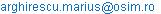To cite this article:

Marius Arghirescu. A Quasi-Unitary Pre-Quantum Theory of Particles and Fields and Some Theoretical Implications. International Journal of High Energy Physics. Special Issue: Symmetries in Relativity, Quantum Theory, and Unified Theorie. Vol. 2, No. 4-1, 2015, pp. 80-103. doi: 10.11648/j.ijhep.s.2015020401.17

Abstract: Through a phenomenological approach using the concept of sub-quantum fluid, the theory argues the possibility of a cold genesis of elementary particles and of fields, explaining the electro-magnetic and the gravitic fields by equations of ideal fluids applied to the subquantum and the quantum "primordial dark energy". The possibility to explain the cold genesis of "dark" photons and of "dark" elementary particles is obtained by a CF -chiral soliton model of lepton, resulted as vortex of „primordial dark energy", respective- as Bose-Einstein condensate of gammonic (e+-e-)-pairs confined in a very strong magnetic field, in the Protouniverse’s period of time. This possibility results by a model of primordial ‘gravistar’ with a self-growing property given by the confining of "primordial dark energy" into "dark photons" and into "dark particles" by a "vortex cascade" mechanism induced by its magnetic field and gravitationally sustained. The supposed primordial "big bang" of the Universe results as a period of gravistars transforming into magnetars, supernovae and into (micro) quasars. The resulted model of expanding Universe gives a semi-sinusoidal variation of the expansion speed. The approach, even if does not propose an enough unitary equation of the known basic fields, it explains naturally the fundamental interactions, by the same basic concept.

Keywords: Unitary Theory, Gravistar, Chiral Soliton, Dark Energy, Dark Matter, CF-Particles, Bosons Confining, Expanding Universe

1. Introduction

In a recent book: "The Cold Genesis of Matter and Fields", of the author , was argued the possibility to explain the fundamental fields of particles by the classic mechanics of the ideal fluids in a galileian relativity, by a simple or composite chiral soliton model of fermion considered as formed "at cold", according to a fractalic „vortices cascade" process and the next structure of the subquantum and quantum medium:

(Ac) – sub-quantum medium; (ms<< mh =

= h/c2; Ss* @ 0; maximal speed: vs »Ö2.c):

-gravitons; (g-etherons): mg = (10-68¸10-72) kg, acting as gravitic field quanta and having contribution as etheronic wind to the genesis of gravitomagnetic quantum-vortices;

-sinergons; (s-etherons): ms = (10-59¸10-61) kg, acting as sinergonic quanta of vortices of gravitomagnetic chiral solitons but also as gravitonic quanta ;

(Bc) – quantum medium, mb ³ mh = h/c2 :

-quantons: mh = h×1/c2 = 7.37x10-51 Kg; Sh*<< ½ħ, acting as quanta of the B- magnetic field and forming the mp     -magnetic moment of fermion; similarily, the pseudomagnetic moment of quanton: mh, results as a sinergonic vortex formed around a quantonic superdense centrol having the mass: mhc = mh , the quanton being -in the theory, the smallest hard-core (pseudo)fermion;

-vectons (vectorial photons): mv = 3x1010mh = 2.2x10-40 kg; Sv=Sv*=½ħ; acting as electrostatic field quanta, resulted as hard-core semiphotons of the cosmic 3K-background radiation;

-vexons; mw ³ 10mv ; Sw=Sw*=½ħ; structured as vectonic chiral soliton assembly, acting as constituents of quantum volume of the elementary particles and of luxons;

-pseudoscalar photons, (particularly-luxons): mf = n×n×mh =2n×mw , Sl =1ħ; acting as electromagnetic radiation pseudoscalar quanta, formed by ‘n’ pairs of vectorial photons: mf = n×(mw-`mw).

The reason of the previous theoretical considerations was based on the fact that the masses of the stable/quasistable free photons or etherons are in the relation:

msk » (Kv)-1×msk+1 ;with: KvÎ (10-9¸10-11);    k³1    (1)

and these (quasi)stable free photons or etherons can be field quanta or pseudo-quanta or/and constituent quanta of the elementary particles with bigger mass.

According to the model, the electron results as a CF chiral soliton with the inertial me-mass with exponential density variation, re = re0×e-r/h , given by the confining of paired and un-paired vexons around a electronic hard centrol, in a quantum volume of radius: re = a = 1.41fm, by the electron’s magnetic moment vortex: Ge = GA + Gm , of sinergons and quantons, the GA -vortex generating the magnetic potential, A, and the Gm - vortex generating the magnetic induction, B = rot.A, by the impulse density gradient of the GA-vortex.

The exponential form of the nuclear potential is theoretically re-found through a cold genesis nucleon model, formed as B-E condensate of paired quasi-electrons (degenerate electrons) and by an Eulerian expression, as being generated by the vortexial dynamic pressure created inside the nucleonic quantum volume.

According to the proposed Cold Genesis Theory (CGT), the vectorial photon and the electron results as vortexes of „primordial dark energy", with confined inertial mass and an evanescent part, the elementary mesons and baryons resulting as Bose-Einstein condensate of gammonic (e+-e-)-pairs, formed by vortexes of "primordial dark energy" in a very strong magnetic field, in the Protouniverse’s period of time.

This possibility results by a model of primordial ‘gravistar’ with a stong vortex of "primordial dark energy"- considered as being composed by etherons and quantons, and with a self-growing property of its kernel formed "at cold", from the vortexially formed leptons, which may be transformed into a mini-"black hole", this gravistar model being similar to the hypothetical "gravastar" proposed by E. Mottola and P.O. Mazur , but relative different.

According to this fractalic mechanism of the particles genesis, it results that in the Protouniverse’s period existed Majorana neutrins which -through theirs vortexial confinement, generated massive neutrins (postulated as components of the Protouniverse also by the Dark matter Universe model) and micro- and mini-black-holes with growing mass and magnetic field.

The possibility of "dark particles" forming by the confination of "primordial dark energy", as "dark chiral solitons", is sustained in concordance with some other theories .

For an unifying model of particles, is argued in CGT the conclusion of a vortexial sub-structure with a radius: a»1.41fm of the inertial mass volume, for all elementary particles excepting the photons and the electronic neutrino.

The considered proto-"dark energy" structure gives the possibility to explain the gravitational and the gravito-magnetic force and field by an unitary theory, based on the similitude between the gravitational force and the electric force, with a similar charge model.

For the weak and the strong interactions, even if the resulted models not results from a more general (unitary) equation of fields, as particular case, these models are based to the same fundamental  model of composite fermion resulted in CGT.

2. An Unitary Theory of the Electric and Gravitic Fields

In accordance also with the charge model of quantum mechanics, according to a classical etheronic theory of fields, , the charge Q(M) of a particle, results as being given by a spheric-symmetric distribution of charge’s quanta: n×mc(rc) around the particle having the electron radius: ra = a, (fig.1), i.e.: ra×r2= ra0×a2, which generates a pressure force over the semi-surface Sx= S0/2 = 2pr02of a pseudocharge qs(m0) approximated as equal with its scattering cross section:          s = p(r0+rc)2» Sx , (rc »0.41r0; r0 = a), given by the impulse density variation : Dpc = pc(r) - pc(-r) = 2n×mcvc :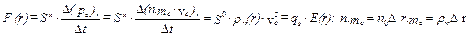(2)

By a gauge constant: k1 = S0e/e = 4pa2/e gauged by the electron with elastic interaction of mc , the electric E-field has the form: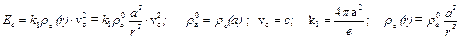(3)

The magnetic B-field is generated when the (pseudo)charge: qs = S0/k1 has a perpendicular v0 = vp×cosq- speed relative to the E-field, (fig.2), according to the impulse density theorem for ideal fluids derived from a Gauss- Ostrogranski relation, which gives the relation for the total electrodynamic force (including the Lorentz force), in the form :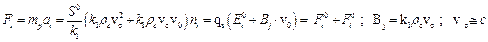(4)

the eq. (4) resulting by the impulse density: pi = rcvc included in the tensor Pik , that is: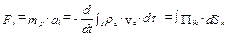(5)

with: k1 given by the equality: ½e0E2(a) =Îv= ½ra0×c2=½(k1-1)E(a), (Îv(a)-the quanta energy) and: Pik -the impulse density tensor: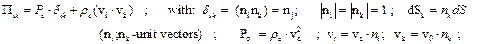(6)

For Pik =constant and ∫dSk = S0×nk , with: S0= 4pa2, for an elastic interaction of the field quanta with the e-charge  and with:  a = e2/8pe0mec2» 1.41 fm,  (i.e. the e-charge in surface),  it results that:  k1 = 1.57x10-10[m2/C]si.

For the elementary electric charge ‘e’ of the electron, the charge sign depends on its intrinsic chirality: ze . Also, a vortex Ge = GA +GB of the A- potential and of the magnetic induction B = rot.A, generates the field lines of the induction B by the gradient of the impulse density: ÑrpA = dpA/dr, which induces xB-vortex-tubes of the B -induction around the vectons of the electric E- field.

The Maxwell’s electromagnetic field equations results in CGT according to eqs. (3)-(4), in a general vectorial form, of a vectorial E- or H- field intensity reciprocal generation :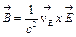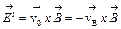(7)

another specific field equation resulting also in a general way from the continuity equation: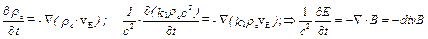(8)

For the electron, according to eq. (4), for r >> rm = 3.86fm representing its Compton radius, the spinning of quantons in the GB-vortex around the e-charge, is realized in conditions of quantum non-equilibrium, according to the vortexial kinetic moment conservation law:

GB = 2pr×vct = 2prmc = ct, and B(r) has the form found by the classic magnetism: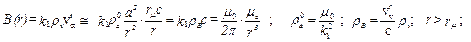(9)

(rB –the density of xB -vortex-tubes ), the magnetic potential resulting in the form: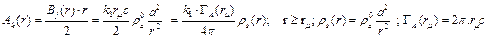(10)

Also, the Lorentz force results of Magnus type - according also to other theories , considering a pseudo-cylinder (barrel like) form of the electron with the high le = 2a and a relative impulse density of the E-field vectons: pv= revvr , generating the B-field according to eq. (4):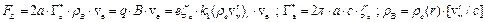(11)

The mp-particle being formed by np quantons having the mh-mass, the eq. (4) is generalisable for the gravito-dynamic force and field, by the relation: S0g = np×½Sh = (mq/mh)×½Sh, (Sh= 4prh2; rh-the quanton radius) , resulted from its penetrability to the g- and s-etherons action and an elastic interaction of the etherons with the S0g –surface.

For the attracted mp-mass and for the gravitic field of an attractive mass M of a particle or of a body, it may be assigned an "electrogravitic" pseudo-charge, qG , respective- by eq. (4), -also an "electrogravitic" field, EG(r,QG), i.e.: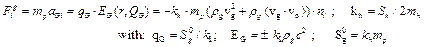(12a, b)

In the expression (12b) of the electrogravitic field intensity, EG, the meaning of the sign: ± is that the electrogravitic QG -charge generating the EG-field is given by an uniform spheric distribution of an etheronic flux with a non-compensated component, i.e. –by the difference between the received etheronic flux and the etheronic flux reflected by the super-dense centrols of the inertial M-mass structure, in the case of an attractive, gravitic M-charge.

Therefore, considering this non-compensated etheronic component as a gravitonic field flux, having the impulse density pg(r)­¯r, the generation of the gravitation force, FN , complies with the Lesage’s hypothesis  which presumes the screening of the mp-mass by the M-mass in report with the cosmic etheronic winds that comes radial-symmetrically towards the M-mass, (fig.2).

The etheronic flux formed by a M-mass with disturbed sinergonic vortex which emits s-etherons, gives an antigravitic pseudocharge, generating a positive, i.e. repulsive EG-field.

For the variation of ρg(r)-density of the gravitonic wind, in compliance with eq. (12) of the electrogravitic qG(M)-charge of the M-mass having the radius r0 and for vg = c , the gravitic force results from eq. (12) as having the form: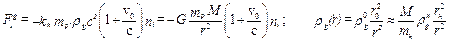(13)

where: ρg0andρgh are the density of the gravitonic flux (i.e.-of the uncompensed etheronic wind) at the M(r0)-mass surface and- respectively- at the mh(rh)-quanton surface.

If the mp-mass represents a photon having the speed v0 = c, the value of the Fig -force, acting as a gravitic type force, results from the equation (13) as being: Fg(r,c) = 2 Fg(r,0), -of a double value comparing to Newtonian static gravitational force, in accordance with the Einstein’s theory of relativity and the astrophysical observations.

A form with lorentzian type term of the total gravitation force Fig , is obtained also in the tensorial theory of gravitation for a weak gravitational field, giving as solutions the gravitational analogs to Maxwell’s equations for electromagnetism, (Lano, Fedosin, M.Agop, N.I.Pallas, , ), the increasing of Fig with the v-speed, being equivalent with an transversal relativistic effect of the gravitational mass growth: Fv = gg×mp(1+b) = gg×mpv, (b = v0/c).

The eq. (13) gives for the G-gravitation constant, the expression: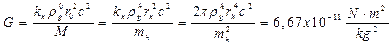(14)

The value of the density rgo of the uncompensed etheronic wind on a black-hole surface -for example, characterizes only the local (not also the intergalactic) etheronic density: reo , because that it results by the speed’s statistic distribution of the etherons emitted by the solitonic quantum-vortices of the elementary particles, i.e. it is proportional with the mass density.

According to eq. (12) and (13), we may use the gauge condition: qGe »e, by the approximation: kr = FN/Fe » rg0/ra0, resulting- by the generalisation: EG = (me/e)×aGi and by eq. (12b), in accordance with eq.(14), the equation: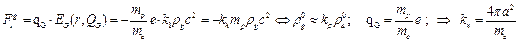(15)

In this case, for an unitary form of the electric and electrogravitic fields, we may obtain a plausible gauge value of kh and of rgh, considering for the electron case the gauge condition: qGe» e, which complies whith the expression obtained by M. Agop , starting from the acceleration of an electron in the field of another: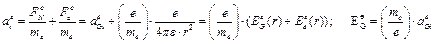(16)

which gives by (15) the gauge constants: kh = 27.4 [m2/kg],  rh =1.79x10-25m; rh= rcM =3x1023 kg/m3; rgh =6.2x10-30 kg/m3 and respectively, by eq.(14): rg0 =1.24x10-29 kg/m3. Also, by eq.(12), it results that: QG = 4pe0GM×(me/e).

If the g- and s- etheron has the same rcM density as the quanton, it results also the size order of the graviton’s and of the sinergon’s radius: rg » 10-31m; rs » 10-28m and the ratio: rs/rg » rh/rs » 103.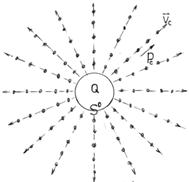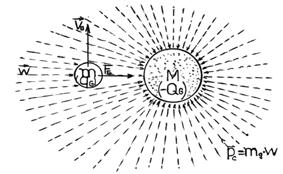Fig. 1. Static type Charg.                                 Fig. 2. The Gravitational Interaction.

By the galileian relativity, it is possible also to avoids the paradoxes of the physical interpretation of Einstein’s special relativity equations, such as the so-called "the twins paradox", by the concept of cosmic ether, by a physical re-interpretation of the Einstein’s relativistic equations such as the equation of speed- dependent mass variation, considering the sub-quantum medium as an ideal fluid with a rs mean density, in which- according to a specific equation for ideal fluids, the acceleration ap of the m0 -particle "falling" is dependent on the "falling" vp- speed because the resistance force of the sub-quantum fluid: F(r,v) = ½Sg0rsv2, (Sg0= khmp), in the form: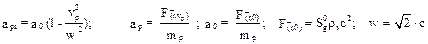(17)

If we consider formally a constancy of the accelerating force F(r,vp), the eq. (17) is mathematically equivalent to a longitudinal relativist effect of the inertial mass variation:

mp*(vp) = mp0/[1-vp2/w2] =m0/b’; with: w = Ö2·c                                                      (18)

According to (18), the etherons may be also tachyonic quanta, with a maximal speed: w =Ö2c.

The expression (15) for the gravito-electric field corresponds also to the Schiff-Barnhill effect which states that in the presence of a gravitational field and in stationary conditions, there is a small electric field generated in a conductor or superconductor: E = -(m/e)×aG.

Combined with the result of eq. (13), the relativist expression of the gravitation force results similar to those of I. Somacescu’s theory, :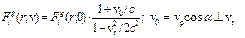3. The Elementary Particles as Chiral Solitons

3.1. The Photon

For explain the wave-particle dualism of the light photon, H. A. Mùnera considered a semi-classic model of vortexial particle-antiparticle pair, which explains the frequency and the repose mass of the photon , , by preonic vortexes of quasi-constant density, formed in the quantum vacuum.

Geoffrey Hunter and L. P. Wadlinger  proposed a solitonic model of photon corresponding to the Einstein’s concept, considered as a confined electromagnetic wave in a volume of an ellipsoid with the length along the propagation axe- equal to the wave- length, λ, and with the photon diameter: df = λ/π.

It may be observed that if the Múnera’s model of photons is dimensioned like the Hunter- Wadlinger model, considering the simple photon as a doublet of two vectorial photons with mutually anti-parallel spins S= ħ/2 and a mean diameter:           dw = λ/p and considering the hard-gamma quanta as a doublet: negatron-positron,  gc= (e+-e-),  with opposed spins and an energy: εγ = hn = 2mec2, it results that the electron may be assimilated with a vectorial (semi) photon, mew, with a Compton radius:  rl = λ/2p, (λ = h/mec).

Because that accordingto CGT, the magnetic field B is given by a vortex of sinergons and of quantons which has the rotation speed: w×r = c inside the pseudo-cylindical volume of radius

rl : ul » 2p rl3 = l3/4p2, from the equality between the vortexial energy: wmf = ½m0H2~ ½mS(wh·r)2and wEf = ½×e0E2~ ½mSc2- given by the translation energy of a spinorial GS vortex of quantons which do not contribute to the inertial mass mv(w) of the photon core, it results for the proposed revised model of photon, the equation: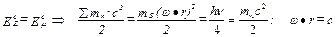(19)

In this case, it results that:

En = 2(EE +Em) = 2msc2 = hn = 2mwc2,

by the conclusion that the spinorial (vortexial) mS - mass of the vexon is equal with its inertial mass: mw (considered in CGT as cluster of vectons with super-dense kernel, contained in a volume uf(rf £a)) which explains its gravitational charge, the photoelectric effect and the atomic electrons transitions.

This equality is argued in CGT by the fact that- for a vectorial photon, by a rw density variation with r-1 –for a pseudo-cylindrical ul -volume or with r-2 –for a spherical ul -volume, the vectorial photon spin results of value: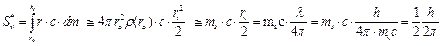(20)

- equal with the value considered for fermions by the quantum mechanics.

The explaining of the equality: ms = mv , may be given by the chiral sub-solitons forming condition  which specifies that the energy EG = mGc2 = 2Em of the mass-generating chiral soliton field , given- in this case by the sinergono-quantonic vortex Gm =GA +GH = 2pr×c, should be double, at least, comparing to the mass energy : Ew = mwc2 of the generated sub-solitons; (E =2Ew).

This condition imply also the conclusion that the particles mass may increase with the speed only by a Gm(v)-vortex increasing but cannot exceed the value 2m0 , (with m0 –the particle rest mass).

The fact that the photon length is -normally: ln =l >2dw = 2l/p , may be explained by the conclusion that the vectorial photon volume ul is a little expanded over the value dw to at least one direction.

Because that some experiments  confirmed the value: l/p for the diameter of the photon section, we take into account the vexon’s volume expansion along the axe x // vp = c; this possibility suppose that the vortexial energy Em of vectorial photon is contained by an ellipsoidic volume with the length : lw =l/2, i.e.: ule » p(l/p)(l/2p)(l/4) » l3/8p, the inertial mw –mass of vexon being closer to the focal point of the ellipsoid which is the first in contact with the pseudo-stationary (brownian) etherons and quantons of the quantum vacuum, of total density: rr =rrc+ rrs, which acts as a relativist wind, deforming the vexonic quantum volume along x //vp , by the sinergonic component: Ps = rrsvp2, until a value: lw = l, (fig.3), i.e.:

pr = rrvp » const. Þ lw = (2rl + Dlw) ~ vp,   (21a)

relation which results in the hypothesis of a relativist etherono-quantonic impulse density: pr = rrvp of quasi-constant value.

By eq. (21a) , the revised model of photon may explain also the photon wavelength variation with the photon’s speed in an optical medium with a specific index of refraction, n, i.e.: vn = c/n Þ λ0/n; (n=2mv/mh = constant), in the form:

Dv = (c- vp) ~ (rr -rr0); lw/ lwc = vp/c;    (21b)

(lwc = lo(c); lw = l( vp); vp » vn )

in which rr0 is the initial density of the etherono- quantonic medium in the quantum vacuum.

Also, because that- in the case of light propagation in an

optical medium in the presence of an intense electric E-field or magnetic B-field the etherono-quantonic density rr of the quantum vacuum is supplemented with a value: Drr ~ e0E2 or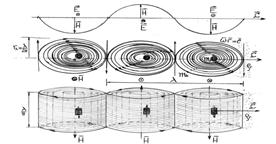Fig.3. Revised Model of Multi-photon, (lp = 1,5 l).

Drr~ m0H2 –according to CGT, it is possible to explain by the model also the Kerr effect  and the Cotton-Mouton effect , of the refractive index changement in an applied E-field or H- field or by the intensity I of the light beam, (the AC Kerr effect), by the hypothesis that the photon speed: vp£ c results by a quantum Stokes force:     FS = KS×hd×vp ,                  (h = rrnr ; nr –kinematic viscosity of quantum vaquum; rr- the etherono-quantonic density), acting over the mp inertial mass of photon and generating a quasi-constant mean deceleration- according to the eqn. (21a), i.e.:(22)

Because that in CGT is considered a radius: rw = k×a with k » (0.4÷1) for the inertial mass of photons, (k » 0.7 for light photons), it results that: d=2k×a, (a=1.41fm), resulting for vand n, that: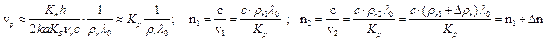(23)

If: Drr » kE×e0E2/c2 and : Drr » kH×m0H2/c2 , (kE ~er ; kH ~mr –proportionality constants depending on the optical medium and on the relative permitivity/ permeability), it results that: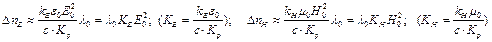(24)

where KE and KH are the Kerr and the Cotton-Mouton constants of the optical medium. So, we obtain a microphysical explanation for the Kerr and the Cotton-Mouton effects.

The decomposing of a monochromatic polarised light beam into two waves: ordinary and extraordinary, in a strong magnetic field, may be also explained by the revised model of photon, considering also the difference of the magnetic interaction with the external B-field of the two vectorial photons which gives the light photon.

Also, in the inverse Cotton-Mouton effect, when an oscillating field passes through a dielectric medium in such a way that it's oscillations are similar to the polarization induced by the C-M effect, then a static magnetic field is created perpendicular to the direction of propagation of light. This effect is also explained by the fact that the Gm-vortexes of light quantum vexons induces parallely oriented vortexes of a H-field in the quantum medium of the dielectric body.

By the model, the photon may be considered un-deformed until a speed:

vpc = 2dpc/l0 = 2l0×c/pl0 = (2/p)c » 0.64c .

The refraction angle may be also explained by a model of double multiphoton.

If the dielectric body has a speed vD //vp* with vp* ¹ vp, if Kn*(vp*) » Kn(vp), it results by eq. (22) that:  vpr = vD + vp* = vp , (i.e.- the invariance of  vpr- speed of light ).

The possibility: Kn*(vp*) » Kn(vp) corresponds to the conclusion that the deceleration ae is equilibrated –inside the dielectric body, by an etheronic wind pressure Pe given by atomic vortexes, which may explain the fact that- when the photon get out from the optical body, it regains its normal speed, c .

3.2. The Electron

According also to CGT, the electron may be considered –in a degenerate form, a semiphoton of a hard-gamma quantum, considered as a pair: e+*-e-* , (degenerate negatron- degenerate positron), which may be splitted in an electron-positron pair in the field of a nucleus.

Because that- according to some other opinions, the charge variation inside the quantum volume of the electron’s inertial mass, me , corresponds to the density variation, in a electron model of confined vexons distributed inside the electron according to a Boltzmann type statistic distribution: re(r) = re0×½y(r)½2 (that characterizes also the mixtures of bosons and fermions), by similitude with the structure of proton, in CGT it results the following substructure of the electron, (figure 4, ) :

-an "impenetrable" supersaturated quantum volume having the radius ai = 0.5÷0.6fm, composed of vexonic layers-in even number for positrons and odd number for negatrons, with paired and magnetically coupled vexons to the radial and the meridian direction;

The qw*-pseudo-charge of vexons of the last layer of "impenetrable" quantum volume, attracts light vexons with oppsed qw* pseudo-charge.

-a charge’s volume which is also of strong interaction quantum volume with the thickness: Da = a –ai, (a=1.41fm), formed by un-paired light vexons: mw*, attracted by the last layer of the "impenetrable" quantum volume and polarized with the pseudo-magnetic moments: mw on the meridian direction by the magnetic lines: xB of the electron magnetic moment, me, (xB vortex-tubes).

The pseudo-charge qw* of the polarised vexons from the strong interaction quantum volume of the electron gives the electron’s charge: e=S(qw*).

- According to the model, the attractive or repulsive interaction is carried through vectorial quanta ("vectons") of the electron e–charge, obtained from the bosonic pairs of the background radiation (from the quantum vacuum), attracted by the Ge vortex of the me -magnetic moment and "splitted" by the mw*-vexons of the e-charge, the mv-vectons having the same q*-pseudo-charge as the mw*-vexons of the electron’s charge being rejected with an oriented spin, forming the E-field, the remained antivectons being absorbed by the mw*-vexons and used for the regeneration of the Ge –vortex .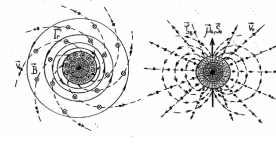Fig. 4. Model of Chiral Soliton Electron.

According to the model, the polarization rate of mw*-vexons giving the electron e-charge, and implicitly- the value of the vectonic flux: Fv(E), are proportional to the impulse density of the Ge- vortex in the strong interaction quantum volume of the electron, by the dependence:

e ~ me(Ge) ~ rm(r)×c2 ; (ri< r £a),        (25)

In accordance with the experiments , it results also the existence of a super-dense electronic centroid (centrol) having the density rm ³ 1019 kg/m3and the radius: r0 = 10-18 m, so –which is a very penetrant particle, being in the model a half of electronic neutrino, with the mass: m0 » 2 x10-4 me =1.82x10-34 kg- in accordance with an experimental result  for the superior limit of the neutrino rest mass.

In the model, the electron’s centrol is characterized also by an intrinsic chirality: ze= ±1, corresponding to a hypothetical helical form which determines the sense of the induced soliton vortex, Ge.

The electron spin results according to eq.(20) by a spinorial mass: ms = me  given by a vortex GS(ul) of  light photons.

The mass of the electron’s quantum volume: ue(a), results according to the eqn: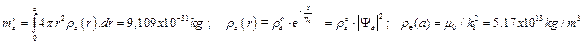(26)

resulting a value: he @ 0.965x10-15 m, that is relative close to the value: hp=0.895fm of the root-mean-square radius of proton charge distribution, experimentally deduced by Ingo Sick  and to the isoscalar magnetic mean radius: rm=0.92fm, given with the Skyrmion soliton model of proton, .

From eq. (26) it results also:

re0 = 22,24x1013 kg/m3.

In conditions of quantum equilibrium: eh(r) = g×(kB/ ħ)×Sh(r), (Sh(r)=2pr×mhc),

(eh – the negentropy per quanton; g =64, ), the sub-solitons forming condition  applied to the electron’s volume, ue, is respected for an identical variation of the densities: re(e), rm(Gm) and rs(GA) -of the inertial mass and of the magnetic moment’s quantono-sinergonic vortex, i.e.:

rs(r) = rm(r) = re(r) = rr(r)/2,          (27)

(rr(r) = r(Gre) =rs(r) + rm(r)) .

In accordance with other soliton models of electron , the stability equation of the Ge soliton vortex may be expressed by the Schrödinger nonlinear equation (NLS) with soliton-like solutions, identifying in this equation the term: kn×|Y|2 , (kn-the nonlinearity constant), with the strong self-potential: Vp(r) of the particle, generated by its Gm-vortex of quantum volume, by the condition: iħ×(y/t) =0 (without contraction or expansion), which express the equality between Vp(r) and the centrifugal potential: Ecf(r) of an infinitesimal vortex volume: due = (dme/rm)r , i.e:(28)

with: kn= -VP0(o); Sm=(dme)r×c×x.

The form (28) of the fermion strong self-potential corresponds to an eulerian attractive force of quantum dynamic pressure gradient: fp =ÑrVp = -due×ÑrPd , generated by a pseudostationary quantonic medium accumulated by the GA sinergonic vortex, having the same density variation and the relativistic c -speed in report with (dme)x .

The same force fp is generated also by the Gm- vortex acting upon a (pseudo)stationary mass having a volume: due .

Because the solitonic nature of vexons, by the eq. (27) it results that the quantum intrinsic energy of electron, which is liberate at electron-positron annihilation, is given by the intrinsic vortexial energy of vexons-induced by Ge-vortex and the kinetic energy of the electron’s magnetic moment:

Ew = ½åemwc2+ ½åmmc(w×r)2= mec2         (29)

in accordance with the quantum mechanics.

By CGT, the previous electron model results as an unifying model of fermion, the mesons and the elementary baryons resulting as composed by cold formed quarks, resulted as clusters of degenerate electrons, i.e: of quasi-electrons, with degenerate mass, charge and magnetic moment.

It results that -in the fermion’s genesis process, in a very strong magnetic field, (as those of a magnetar), at quantum equilibrium, when: Gc =2pmcc, the genesic QG- quantum potential resulted by the formation of the sinergonic GA -vortex and acting over a quanton moved to the Gm--vortex line: lr = 2pr (r£a), compensates the quanton’s centrifugal potential: QG = - Ecf = -pc2/2mc, the nature of the QG potential being a magneto-gravitic genesic field, acting by a pseudomagnetic (sinergonic) BS- induction in report with the mc-pseudomagnetic moment of the quanton. According also to the eq. (4) of the B-induction, it results that:

QG = -mc×BS(r) = -mc×k1×rs*c = -pc2/2mc = -h/2 = - Ecf  (30)

The dynamic equilibrium of forces, for rs= rs0e-r/h;         h » 0.96fm -in accordance with eq. (26), gives:

FG=ÑQG= (-1/h)·QG = (1/h)·h/2 = mhc2/r= h/rl; Þ rl =2h»1.92fm >a                           (31)

For the electron cold genesis, the eq. (27) resulted from the chiral sub‑solitons forming condition , impose- by the relation: 2pa3×r0a = me , (specific also to a proto-electron), the condition: rs*ra0 = 5.17x1013 kg/m3, resulting: mc→1.36x10-46A×m2; BS→2x1012 T.

A consequence of the chiral soliton model of charged particle is the conclusion that- at the fermions vibration under energy shocks, the vexons from the particle’s quantum volume are easier destroyed and their vortexial structure is disturbed, decreasing the elastic character given by the photon interaction with vexons of the e- charge’s surface; in this case, photons which in the unperturbed state are reflected, can penetrate the quantum volume.

Through the Gm quantum vortex of the particle, these photons are converted, inside the impenetrable quantum volume, into vexons with bigger mass which may be emitted as stable-bounded vexon-antivexon bosonic double pairs: 2(mw-`mw), having a null prequantum spin, (i.e- a scalar radiation quantum), under the action of the soliton quantum-vortex of the m magnetic moment, according to equation:

n.εn + mpc2®(Ev)® mp*c2 + εW ; n.εn @ εW ; Ev ³Ev0 = εW/Kv                                            (32)

where en; ew –represents the energy of the captured photons and, respectively, of the emitted scalar quanta and Kv is a constant which can be also of over-unity value, in accordance with the energy conservation law, the eq. (32) explaining in this case the results of some experiments, such as the kinetobaric effect , the experiment of photons‑electron interaction, (Stanford, 1997, ), of g-rays emission by interaction with green laser pulse and the observations of γ-rays emission generated by thunderstorm, (italian group, 2000, ).

Another consequence of the chiral soliton model results by the fact that the dependence: eh(r) ~Sh(r) ~r , for the electron, shows that the vortexiality gives negentropy, i.e.- an energy which may be converted as in the case of some functional „free energy" devices using long life magnets, (magnetic motors, etc.).

3.3. The Proton Model and the Nuclear Force

In CGT, the proton is a (Np)-quasielectrons protonic cluster, with quantonic Gm*-vortices of paired degenerate electrons, induced by the sinergonic GA*=GAe vortices around each electronic centrol, with the degenerated density variation of the Gm* quantonic vortex of me*-magnetic moment, corresponding by eqs.(25)-(27), to a degenerate charge: e* = 2/3e, (characterizing the quark’s charge), i.e., to:

rm(a) = 2/3re(a) = 3.44x1013 kg/m3, which corresponds by eq.(26), to: h* =0.755 fm, the degenerate quasielectron mass resulting by eq. (27): me*= 0.8722×me = fd×me , (fd –mass degeneration coefficient).

The proton’s e+-charge results in the model by an attached positron, placed axially (at r+> h*) in the strong interaction quantum volume.

In this case, the neutral proton cluster is formed by : Np=1835.1/fd @ 2104 paired quasielectrons, according to the model, i.e. by degenerate gammonic pairs (e*+- e*-), confined „at cold", in a very strong magnetic field and forming a Bose-Einstein condensate of gammons, according to CGT.

The loosed part of electron energy: Dee = ( me - me*) @ 0.13mec2 = 65.3 keV, in the bound degenerate gammon formation process, has the signifiance of a binding energy, as in the case of the deuteron’s forming.

A small repulsive static type charge of quasielectron centrol, given by a sinergonic static pressure, impede the confination of the proton’s kernel until a density: rk = rm .

The virtual radius: rmn, of the proton mp-magnetic moment, compared to the electron, decreases when the protonic positron is included in the Np cluster volume, from the value: rme = 3.86x10-13m, to the value: rmp = ri = 0,59fm, as consequence of the increasing of the impenetrable quantum volume mean density in which is included the protonic positron centrol: m0 , from the value: re , to the value: `rn @ fd ×Np×re , conform to: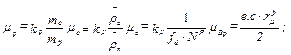(33a)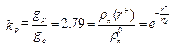(33b)

in which: kP -the gyromagnetic ratio; `re; `rn – the mean density of electron and nucleon;

r + -the position of protonic positron centrol in report with the proton centre.

The interpretation given by eqs. (33) of the particle mass-depending magnetic moment variation, explains also the fact that- when the proton is transformed into neutron, the emitted positron regains the me magnetic moment value of free state, by the negentropy of the quantum and the subquantum medium, given by quantonic and etheronic winds.

The virtual radius of the proton magnetic moment: rmn = 0.59fm- resulted from eq. (33a), may be considered approximately equal to the radius of the impenetrable nucleon volume, of value: rmn @ ri(mni) @ 0.6fm- used in the Jastrow expression of the nuclear potential, , by the conclusion that the impenetrable nucleonic volume being supersaturated with quantons, limitates the radius decreasing of the         Gmp =2prmc -quantonic vortex, at the value: rm0 = ri.

-The value mN = mc/1836 for the nuclear magneton gives -by eq. (33), a magnetic moment radius: rio = 0.21fm, that represents the Compton radius of the proton, close to the experimentally deduced proton core radius rm, (0.3fm-) and to the experimentally deduced proton quark radius, .

The relation (33b) also gives: re+ = 0.96 fm for the protonic positron axial position.

Because that–for the electron CF-model case, the vexons of electron surface has a degenerate Compton radius approximate equal with the electron Compton radius: rwe @ rme, it results by eq. (33), for a vexon which is in the proton’s surface, (rw @1.4fm), that: rwn@ (rme/1836)×e1.4/0.93=0.946fm, so: rma = a+ rwn= 2.35fm, rma being the virtual radius of mp, for which: Gmp = 2prma c.

The resulted value rma ®2a, is conformed also to a pre-quantum soliton model of atom, (which degenerates in the Bohr-Sommerfeld’s model at T>0K), for which:

ve(r)/c = Ö(2a/r) ; ve(r0)/c = Ö(2a/r0) = 1/137;

(r0=0.53Ǻ; ve(r) = ve(r0)/n; rn= n2r0),

by the conclusion that the sinergonic GA-vortex of the protonic positron gives the ve(r)- speed of the atomic electrons by the action of a tangent force: FA(r), given by the impulse density: ps(r) = rs(r)×w, (w=Ö2c) of the GA vortex, in a dynamic equilibrium with the advancing resistance force: FR(r), given by a density: rR of an equivalent pseudo-stationary sinergonic medium, without photons emission.

This solitonic model of atom allows also the explaining of the electron transition on under-fundamental level (n = 1/2) in the hydrogen atom, (for hydrino atom), observed in some experiments of cold nuclear fusion, , by the hypothesis that the quantification of the electron number of an energy level: N(n), corresponds to a superficial charge density se of constant value for an energy layer considered of quasi-cylinder (barrel- like form) , with the height: ls and quantified  radius, re = n2r0 , i.e.:

N(n) = Q(n)/e = (σe.2πre ls )/e = 2n2; Q(1)= 2e; ro = e/(σe.π. ls); re = n2.ro                         (34)

According to the model, the transition on under-fundamental level (n = 1/2) is particular to the hydrogen atom, by the condition Q(1/2) = e, (H-atom having a single electron), condition which gives a radius for the under-fundamental level orbital: ro* = e/(se.2p.ls ) = ro/2.

For other atoms, with bigger mass, the transition on under-fundamental level: (n=1)®(n’=½) results as possible by stimulated electronic transition, by which may be obtained also „mascons", i.e- metals with concentred atomic mass, (bigger density than the normal density), according to the model.

At the proton’s level, the superposition of the (Np+1) quantonic vortices: Gm* of the quasielectrons magnetic moments, generates-inside the volume with the radius:                    rma =2.35fm, according to the superposition principle, a total dynamic pressure: Pn = (1/2)rn(r)×c2 having a variation according to eqs. (26) and (28) and acting over the impenetrable nucleonic volume ui(ri;mni) » 0.9 fm3 of another nucleon, (the rest part being penetrable to the quantons action).

This dynamic pressure Pn , generates a nuclear potential and force, according to eqn.: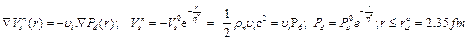(35)

the proton density in its centre resulting: rno =(Np+1)×reo =2105×reo = 4.68x1017kg/m3, (re0=22.24 x1013 kg/m3) and with: h* =0.755fm, the potential well resulting: Vso = -118.4 MeV.    Thus, by eq. (35) is theoretically re-found the form of the exponential nuclear potential, with specific constants.

At the distance d @ 2 fm between deuteronic nucleons (considered as the dimension of the nuclear potential well), it results from the relation (35) that the scalar nucleonic potential Vsn(r) has the value: Vsn(d) = -8.37 MeV- value which corresponds to the known mean binding energy inside the stable nuclei: -7.5….-8.5 MeV.

The mesonic theory of the nuclear force results -in consequence, by our interpretation of the eq. (33), as formal.

According to eq. (35) it results also that the deuteronic self-resonance decreases the value of scalar nuclear potential, .

The sinergonic dynamic pressure: Pds(r) of the GAn vortices of (Np+1)-protonic cluster, generates a scalar gravito-magnetic potential Vsn(r) similar to the nuclear potential but acting upon a volume: ucn @ mp/rM = 1.67x10-27/8.8x1023@ 1.9x10-51 m3given by the quantonic and electronic super-dense centrols of the proton mass, so- of ~100 times smaller than the nuclear potential, but contributing to the stability of the proton’s chiral soliton.

However, the magneto-gravitic potential is of an important value for "black holes" and for "magnetars". For r > rma, by eq. (35) it results that the magneto-gravitic potential generated by an elementary particle over another particle having the mass mp, has the expression: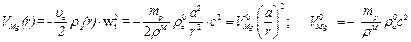(36)

3.4. The Neutron Model and the Weak Force

Complying with the CF proton soliton model, the neutron results in the theory conforming to a Lenard-Radulescu dynamid model, (Dan Radulescu, 1922, ) , as being composed by a proton center and a negatron revolving around

it with the speed ve* < c, at a distance re* £ a at which-

according to eq. (33), it has a degenerate meS magnetic moment and Sen-spin, (figure 5).

The revolving of the neutron negatron, generates a negative orbital magnetic moment: meL, the neutron’s magnetic moment resulting by: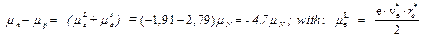(37)

The neutronic negatron orbital rotation takes place under the action of the dynamic pressure: ½×ρμ(re)c2of the Gmn vortex quantons forming the proton magnetic moment mp and inside the quantum volume having the density rn(r), so we can consider the equilibrium relation of the dynamic pressures, acting over a revolved degenerate negatron area: S’ @ pai2, i.e.:

ρμ(re*)c2 = ρn(re*)ve2; Þ ρμ0c2» fd×ρn0ve2, fd=0.8722                                            (38)

With: rm0 =re0=22.24x1013 kg/m3; rn0 =4.68x1017 kg/m3 , it results that: ve= 0.0233×c = 7x106m/s .

Also, by eq. (33) applied to the electron magnetic moment’s degeneration at the incorporation of the neutronic electron in the protonic quantum volume, it results that: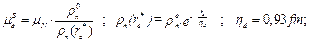(39)

Thus, from (37), (38) and (39) it results:

re* = 1.41 fm; meL @ -0.1563mN; meS @ -4.554mN ,

which leads to the conclusion that the neutronic negatron has the impenetrable quantum volume uie with the centrol positioned in the surface of protonic quantum volume: un, (figure 5), comparative with the positronic proton for which the eq. (33) in which:

me+ =mp=2.79mN , gives: re+ = 0.96 fm.

The neutron’s spin and the revolving frequency of the neutronic negatron around the proton centre results by the relations:

ne = ve/2pre = 0.79x1021 Hz ;  Sen= meS×(me/e) =0.0025 ħ, (ħ=h/2p) ,                                         (40)

-in accordance with the equality between the spin of proton and those of the neutron, (Sn=Sp=½ħ), resulted in the quantum mechanics.

So, by eq. (33), our model of CGT solve the classical problem of the nucleon’s spin and of the magnetic moment values, problem which determined the abandonment of the classical nucleon models presuming incorporate nucleonic electron(s). For the anti-neutron, the model imply the rotation of a positron around of an antiproton.

The continuous energy spectrum of the b radiation at neutron’s transformation, corresponding to a speed ve of the β-electron up to 0.7¸0.92c, is explained- in accordance with eq. (34), (35), through the acceleration given to the b-electron by the vortex Gm of the protonic mp- magnetic moment, the given energy depending on the angle of the electron’s initial impulse, q (pb,rp).

The fact that- according to the neutron "dynamide" model, the protonic positron coexists with the neutronic negatron inside its quantum volume until the neutron’s transformation, may be explained by the model through the hypothesis that the difference of approximate 2.53 me between the neutron mass and the proton mass is given by a binding g*-gammon , called "s-gluol" in our model, which has the intrinsic energy:

Îs = 2me*c2 @1.74mec2 @0.889 MeV      (41)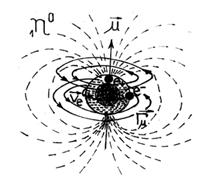Fig.5. The Neutron Model.

The reaction of neutron transforming, :

0ne ® 1pr + -1β + `ne + Qk(780keV)                   (42a)

results from the proposed model, in the form:

(Mn* + g0 + s ) ® (Mn* + e+) + e- + `ne + Îs(889keV); (Mn* + e+) =1pr            (42b)

as given by a gammonic dynamid: g0 = e- + e+, splitted by the transformation of the s-gluol.

According to the model, the electronic pair: negatron-positron of the neutron, represents a gammonic metastable state: g0 = e- + e+, attached to the particle cluster M* with rotating negatron.

Also, the centrol couple having the mass: 2m0, of the disintegrated s-gluol, is emitted under the form of an electronic antineutrino, having the approximate superior limit of repose mass: mn(ne)  =2m0 @ 4x10-4me =3.6x10-34kg, , by the action of the local quantonic and sinergonic pressure of Gmn and of GAn vortexes, with the speed v ® c .

Also, because that the GAn –vortex generated by the protonic positron, contains also tachyonic sinergons, with w ®Ö2c (according to CGT), it is possible also neutrino speed: vn®1.1c, as those observed in the OPERA experiment , according to the model, .

The neutrino model of the theory is complying with the Majorana model, which considers the neutrino as a superposing of two Majorana fields having equal masses and opposed CP parities and with the fact that the neutrino is a very penetrating particle.

According to the model, there are possible also the transformations:

e+ + e- ®g® ne + DÎg(~1MeV) ; p0 + g® p0 + ne + DÎg                                                                (43)

that  may occur  at high energies of matter compression or by collision with relativist mesons or baryons, with the releasing of the quantonic but also of the sinergonic energy of g-quantum:

DÎg = (2me-mn)c2,  (mn -the neutrino rest mass).

Also, it results that similarly, the protonic degenerate electron that gives the proton charge and has an axialli position at re+ = 0.96 fm, is linked by another s-gluol, which explains similarly the β+ decay:

Energy + p+ n0 + e+ + νe                  .,

of proton’s transforming.

The escape of β-electron from the nuclear field results in conditions of the neutron self-resonance with a intrinsic vibration energy, Ene, of the neutronic electron, induced by a vibration energy of the neutron Evn(d), which satisfy the conditions:

Enn(d)+Îs®En0(d) = 2.226MeV;              (44a)

Ene®mec2=0.511MeV                   (44b)

In this case, the natural equivalent of a W± -boson mediating the weak interaction of b-disintegration, used in the quantum mechanics standard model, is the „weson": w - = (s + e-) ,     which generates the beta disintegration in the form:

w -® e- +`ne + Îs when: s ® ne + Îs ,   (45)

The reciprocally opposed quantum helicities of the negatron and positron, remarked in the b- and b+ disintegration (Wolfenstein ), are explained in the theory by the spin dependence of the ze-intrinsic chirality of the m0-electronic centrol which- by its considered helix form, passing through a quantum and sub-quantum medium, determines the orientation of the electron spin-parallel or antiparallel with the impulse direction. by electroncapture: 1pr +e- ® 0ne + ne , may be explained similarly by the conclusion that the captured negatron and the protonic positron forms a metastable gammonic state: g0 = e- + e+, which is transformed into an ne-electronic neutrino, (eq. (43)).

Also, according to the model, at high temperatures as those of a supernova, by the perturbation of the nucleonic structure by the vibration of its kernel, the e+ gammonic positron may be not retained by the neutronic cluster Mn* and the neutron may be transformed with a temperature-dependent probability, by gammonic emission:

(Mn* + g0 + s ) ® Mn* + g0 + `ne + Îs(889 keV) (46)

This theoretical conclusion may explain the cosmic poulses of gamma radiation detected as coming from the direction of Oort cosmic cloud  and resulting by collision of nuclear components-phenomenon not enough understood .

By eq. (46) the phenomenon may be produced also by pulsatile contraction of the volume of a supernova or of a neutronic star.

The particle deconfination may be explained also by the CF particle model as a result of the vibration energy: ev, of n current mass quarks, structured as cluster of quasielectrons .

Considering the lepton m+ , having a lifetime:

tm =2.2x10-6sec. , as a single-particle cluster, and taking into account that the majority of baryons (considered with three quarks in the cluster M* sub-structure) have a lifetime value: t @ 10-10 sec. and the majority of mesons have a lifetime of approximately 10-8 sec. at the ordinary temperature: T@ 300K of the medium which contains the particle, the lifetime of the elementary particles results -by the dependence on the destroyed mass: tk ~1/DmP(T), induced by the total intrinsic ev-vibration energy of the M*-cluster, according to an semi-empiric relation of approximation: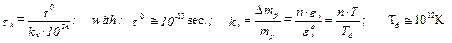(47)

in which: ec0= kBTd @ hnc0 represent the critical phononic energy of particle vibration which determines the quark deconfination, (at: Td »TD = 3x1012K, according to the experiments ).

As a consequence of eq. (47), when a particle pass with the v- speed through a quantum medium of the space, the dynamic quantum pressure generated in a relativistic way by the quanta and subquanta of this medium, has a cooling effect for the M*- particle cluster, (which explains also the existence of polarized quantum vacuum bosons as metastable particles), according to the equation: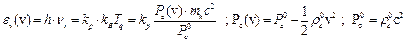(48)

which is equivalent with a relation for the intrinsic quantum temperature variation, of the form:

Tq(v) = Tq(0)×(1-v2/2c2) = Tq(0)×b’; kB×Tq = mhc2                        (49)

similar to the Einstein’s relation: T=T0×b, but with b’ in the classic form (18). Also, by (48) we have: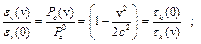(50a)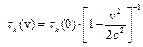(50b)

The eqns. (49), (50) explains also the lifetime increasing for relativistic m±- mesons or other relativistic particles with: v ® c, the eq. (92b) being mathematically quasi-equivalent to the einsteinian relativistic relation used by Rossi and Hall, , but naturally obtained.

3.5. The Deuteron’s Self-resonance and the Quasicristallin Nuclear Model

In the deuteron’s case , the experiments evidenced a binding energy: DE(d) = -2.226 MeV for the real deuteron having parallel nucleonic spins and of about –0.07MeV for the virtual deuteron, having anti-parallel nucleonic spins, .

Comparative to the binding energy value:

Vn(d) = -8.4 MeV, (d = 2fm), of the un-perturbed deuteronic state from stable multi-nucleonic nuclei, the value DE(d) = -2.226 MeV indicates, by eq. (35), a decrease of the quantonic dynamic pressure: Pd(r) = 1/2rc(r).vct2 in the composite chiral soliton of the Np protonic cluster.

This decrease is generated by the decrease of the rma - radius of the exponential part of quasielectron chiral soliton, for the Np-cluster, at a value: rmc < rma =2.35fm, as consequence of the perturbations caused by an intrinsic vibration inside the deuteronic nucleons, with an energy Ev which decreases also the value of nuclear potential well: Vs0, in accordance with eq. (35), by the energy: ev = Dmwc2 of destroyed vexons, to a value:

Vs0*= kv*×Vs0 ~ Ev(d,lv) ; (kv*£1),

with: Ev(d,lv) the mean vibration energy permitted by the vibration liberty: lv= An , i.e.: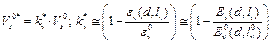(51)

in which ev0 ; Ev0(d,lv0) represents the critical values of ev and Ev(d,lv) which cancel the attractive potential: Vs*(d).

Because that the mass defect: DmD = (mp+mn-mD) @ 2.23MeV/c2 results at the deuteron’s formation as destroyed vexons mass: ev0/c2,which corresponds to the binding energy: DED, it results that:

Ev0(d,lv0) =½ mpvp2(d) = ev0 =DED = 2.226 MeV.

This conclusion is in concordance with the Onsager’s observations regarding the circulation value decrease for a super-fluid perturbed over a critical value. From an energetic point of view, the effect of the vibration energy: En £ DED may be explained by the contribution of the nuclear potential Vs(d) to the deuteron self-resonance state, through an alternately „destruction-regeneration" mechanism of the deuteron state.

According to the model, (fig. 6), simplifying, we may approximate also that the initial value: V(rma) of the potential well is recovered by the negentropy of the etheronic winds at the distance-limit between proton and neutron: rd = d + An for which the nuclear potential, given by eq. (35), has the approximate value: Vs(rd) = DED = -2.22MeV, i.e: rd @ 3fm and An = ln* = 1 fm, according to: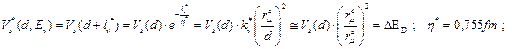(52)

For: rma = 2.35fm,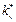=0.72, lv*=1fm, it results from eq.(52):              rmc×Ö@1fm;  rmc @1.2fm  and:  Ev(lv*) = 0.66MeV.

This theoretical result complies with the conclusion of quantum mechanic’s deuteron model, which shows that- on average, the deuteronic nucleons are found outside the limits of the potential well having the length: dd = 2fm, the probabilistic deuteron radius being: RD = 4.32fm, .

-In the virtual deuteron case, the nucleons having anti-parallel spins, the neutronic negatron revolves as in its free state around the proton center of the neutron, passing periodically with the frequency: ne = 0.79x1021Hz between the two deuteron’s protonic centers, (figure 6).

Each time when the neutronic negatron passes between the two deuteron protonic centers with a magnetic moment parallel with the proton’s magnetic moment, it intervenes against the proton with a repulsive magnetic potential: Vmn(dd/2) @ 0.3MeV .

The deuteron’s protonic centers, as a consequence of the induced deuteron’s self-resonance, are thus re-separated to a distance: rd’= d + Av*’with Av*’> 2ri , which determines –in accordance with eq. (52), a maximum decrease of the degenerate value: rmc at the value: rmp = 0,6fm, which corresponds at : lv*= Av*@ 2 fm, the scalar nuclear potential decreasing at a minimal value: Vs*(d;lv*) @ -0.6 MeV which is canceled by the remained nucleon’s vibration energy, explaining the fact that the deuteron with anti-parallel nucleon spins is a virtual state .

In a conventional very simplified form, the spin-dependent nuclear potential may be expressed- in accordance with the resulted model, in the form: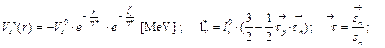(53)

with: Vs0=118.4 MeV; lv @ Av ; lv0(Ev) @ 1fm -for the deuteron; (lv(Ev=0) = 0 ).

The deuteron model of quantum mechanics considers also a self-resonance vibration mechanism for deuteron, but in a different way, explaining the decreasing of the deuteron’s binding energy to the value: ED = -2.226MeV by considering a reciprocal vibration: Ev @ 20MeV, , of the deuteronic nucleons - value which is in a relative discrepancy with the value ED of the binding energy.

Comparative with the plastic interaction of nucleons, with Av ® 0, when the vexon’s energy: Dev(Drn0) of the nucleon superficial destruction is emitted, (Dev = Dmn.c2), in the deuteron’s case, this energy is a nucleon’s re-separation energy, which-by partial absorption, is re-used for the regeneration of the nucleon’s mass and vorticity, by the GA* -vortices.

Conforming to the solitonic CF nucleon model and to the observations regarding the nuclear stability that shows a maximum stability for the even-even nuclei, the pre-quantum nuclear model, of T®0K, results as a quasi-crystalline cluster having nucleons coupled in deuteronic pairs, and corresponding also to the a-particle cluster model, to the "nuclear molecule" model and to the extreme-uniparticle type model, , the stable nuclei, with a "magic" number of protons or/and neutrons: 2; 8; 20; 28; (40); 50; 126, being- in the model, symmetrical quasi-crystal forms, resulted from the superposition of square root forms with an integer n2-number of a particles, having 2n2 protons :

Z = S(2n2), (n = 1.2….7, figure 6), and with tendency to a minimum deformability: 2; 2x22 =8; (2x32 =18); 18+2=20; 20+8=28; (2x42 = 32); 2x52=2x32+2x42=50; 50+32 = 82, or of quasi-stable triangular forms (10Ne) or hexagonal forms (19K) completed with additional neutrons, for Z >20.

The 82Pb208 nucleus corresponds to the initial form: 104N208 (Z=2(42+62)) in which 22 protons was transformed into neutrons by b--emission giving Z = 82, according to the model. Similarly it may be formed a nucleus with A = 4(52+72) =296 , with Z=112¸114, (close to the predicted stable form 114/298).

The weakly bound excedentary nucleons or alpha-particles formed from the valence nucleons, are revolved around a nuclear quasicrystal, (Lonnroth, ), as in the extreme-uniparticle (Schmidt, ) model, by the action of quantonic GmN-vortex of the nuclear magnetic moment, which explains also the nuclear centrifugal potential.

A quasi-crystalline nuclear structure was evidenced by experiments of a-particles scattering on heavy nuclei, (W.Bauer, ), The nuclear fission is explained through the deuteronic self-resonance mechanism of weakly bound nucleons, which decreases the nuclear potential in regions with incompleteness of the quasi-crystal network or with exceeding nucleons, according to eq. (53).

Through the same relations (46), by the deuteron’s self-resonance mechanism and without the hypothesis of exciting energy concentration on a single nucleon, it is also possible to explain the following:

-the compound nucleus transformation mechanism by excitation with particles having low energy, up to 2MeV, as in the case of Be9 which can be transformed with a gamma quantum of only 1.78MeV, even if the binding energy, given by its nucleons, is 58 MeV;-some reactions with thermal neutrons (having some tens of eV) as in the reaction: -Li7+H1 ® Be8+2He4+g, generated with only 125eV proton energy, or generated by thermal neutrons in typical reactions (n;a), such as the reaction: B10+n ® Li7+a, even if normally there are necessary neutrons with 0.5…10MeV; ;

-nuclear transformation with particles having only 1÷2MeV: Ca(p, n)Sc; Al(p, a)Mg;

-the super-asymmetrical nuclear fission, .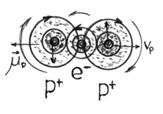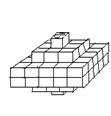Fig. 6. The deuteron and the Quasicristal nucleus.

3.6. The "Dark Matter" as Bosons of the Quantum Vacuum

According to CGT, in the strong interaction of particles, the interaction energy generates real (q-`q)-pairs of quarks, considered in a quasielectrons cluster model, with the charge given by an un-paired quasielectron (+2/3e) or by a quasi-positron and a degenerate electron, (-1/3e), these (q-`q)-pairs resulting from the polarized quantum vacuum bosons, (named "zerons" in CGT), considered as particle-antiparticle pairs with self-resonance realised according to the incertitude relation: DEv×Dtv @ ħ; (Dtv; DEv-the vibration period and energy), the "darkness" of the vacuum Mb-boson being given by a very low self-vibration, Dxv<<Av, with Av = ħ/mzc , ( by T= En /kB ®0K), as in the eqn:

nm + pr + Qi ® nm + pr + 2(m1+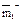) + (m2+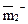) ® nm + pr + p+ + p- +p0; (m2+) = mZ2

So, the hypothesis of neutral Z0 boson or Higgs boson of Q.M. is not strictly necessary for explain the particles cold forming and theirs interactions, according to CGT, the generating of particles with bigger mass than those of particles that enters in reaction being explained- in our theory, by the decomposing of quantum vacuum "zerons" of mz -mass and  xr = a radius, in real (q-`q)-pairs, by the Qi - interaction energy, considered also in quantum mechanics, when: Oi » Eq = mzc2.

These "zerons" of ‚quantum vacuum’ are- in our theory, a classic equivalent of the bosonic background of "dark matter" and may be considered as bosonic mz -particles with self-resonance, (oscillons), with a phononic intrinsic vibration energy of paired quarks, given by: En @ (Dp×Dxv/Dt) < Eq, (Eq=mzc2; Dxv £ 2a; Dt; pDxv -the self-resonance period and amplitude), which explains the existence of pseudo-virtual paired quarks and fermions in the "quantum vacuum".

The cold formed quarks, have- in CGT, a current mass which gives the particle’s rest mass according to the sume rule, .

It results also by CGT the possibility of exotic particles cold forming as hexaquarks or nine-q clusters and the quark®fermion transforming, (q+²/³®p+1), at T>>0, by the relative detaching and moving in the quark interaction quantum volume (Da), of the un-paired quasielectron e* which gives its charge e*=±⅔e and which is auto-transformed in this case in degenerate electron with e-charge (and degenerate magnetic moment and spin), i.e.: q±®p±1; (e®e±1, by the quantum  medium  negentropy).

4. Implications of the Theory in Cosmology

4.1. A phenomenological Model of Expanding Universe

According to the CF-model of the theory, it results also that the fermions entropization at high temperatures with partial destruction, generates a temperature-dependent mass decreasing and a pseudo-antigravitic field of a Qa- pseudocharge (eq. (43)), having the form (10) and a value proportional with the particle vibration energy: ev= kBT.

This theoretical conclusion may explain the observed temperature-dependent gravitational mass decreasing for which Shaw and Davy  obtained a relation of temperature-dependent gravitational force with the form:

FG(T) = F0(1 -aT); F0 = -G×(M×m)/r2             (54)

a = 1/TG = 2.0x10-6 [K-1], (TG = 5x105K).

Because that the quantity of destroyed intrinsic vexons is proportional with the vibration energy: Dmpc2» ks×ev = ks×kBT, (ks<1 constant), it is logical to consider a temperature-dependent decreas­ing for the inertial mass for all particles, in the form:(55)

The value TN »1013K results from the energy necessary to nucleonic kernel: Npm0 , for penetrate the proton impenetrable quantum volume, at speed v0® c, in a classic expression permitted by eq. (18), which gives an approximate value: E0 = ½Npmoc2@ 0.11MeV, that is obtained by the proton’s vibration with an energy:

Î0p = ½mp.c2 = 0.47GeV

and a critical fre­quency of its destruction:

nc0 = 1/tc = c/a = 2x1023Hz .

The energy which must be given to the proton for its destruction is obtained by the relativist expression of mass: mpr = mp/b’, given by eq. (18) with v0®c, and corresponds to a proton energy : ÎRp= ½mpr.c2 =2Î0p= mp.c2= 0.94 GeV- equal with its intrinsic energy, which explains the proton destruction mechanism in concordance with the inferior limit of the proton’s destruction energy obtained by the quantum mechanics.

The quasar’s energy generated by nucleon’s mass®energy transformation, by a nuclear temperature: TN = ÎpR/kB » 1013 K , is more plausible than those imposed by the Big‑bang model of Universe, (1014K).

This phenomenon indicates as plausible an open-type phenomenological model of expanding Universe, according to CGT.

For a model of the Universe evolution, the Hubble’s law of the cosmic expansion: vR =H×R, which is confirmed for the case of our cosmic time: tL and our location from the Universe centre: RL, results in CGT as a particular case, by the existence of many repulsive antigravitic charges of ultra-hot stellary sources, with a mean density: ra(R, tE), the total mean gravitic charge density: rGt= (rM + ra)R, being given by a gravitic and an anti-gravitic charge density of the matter.

Considering also a Macronucleus of Universe with a R0 radius having a macro-black-hole with a Macro-vortex around it, which has the density variation proportional with the mean matter density: rm(R) ~ R-1, we may consider also a variation of the etheronic pressure: Pc(R) ~ [R-1¸R2] with the R-distance from the supposed Macronucleus, specific to a magneto-gravitic pseudo-vortex with similar density variation as the matter density, the gravity G- constant depending on the quantum pressure: Pc(R) by the etheronic density, rG0, according to eq. (26).

Thus, close to the limit R = Ru - considered as the structured Universe radius, the gravity force and the quantum vortices intensity becomes too weak for forming or conserving vortexial structures. In this case, we may consider that the zone: DRu £ (3Ru/4 ¸ Ru) represents a zone of "stellar cemetery" (S.C.) in which the stellary structures disintegrates at the distance: Rd³ 3Ru/4 and that the protons and the neutrons disintegrates at the distance close to R= Ru -as a consequence of the decreasing of the nucleonic strong interaction potential, according to the CGT’s pre-quantum chiral soliton model of particle.

This conclusion corresponds- partially, to the Universe bubble model, (i.e.-limited Universe).

The expanding of an ellipsoidal quasi-flat Universe with a mass: MfRu ~2R0×pRu2×ru for which the local mean matter density is: rm(R)~R-1, may be approximated according to the Poisson’s equation as being equivalent with a deformed spheric Universe with rm’(R)~R-2 having the same mass with it for each R- radius, (fig. 7), by the equations: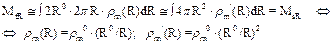(56)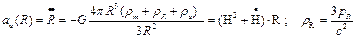(57)

with: rR; pR - the space radiation density and pressure , (mainly- of 3K), rm- the mean matter density; ra –the mean antigravitic charge density and: v(R) = dR/dt = H×R.

The eq. (57a) is classically equivalent to eq. Einstein-Friedmann for the flat Universe (k=0) with negligible matter pressure, pm, by: úraê= 2r*L, (r*L -the " dark energy" density,  ra (R) ~Tu(R), (Tu- the mean Universe’s temperature- eq. (55)), i.e. -with the equation: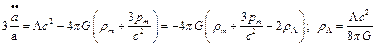(58)

According to eqs. (57)+(58), the Universe expansion is obtained by the antigravitic charge of the total matter, of density rm , but given by the part of the observed matter, for which:

WM = rM/rc @ 0.05, (the rest being "dark matter") and with WL =rL/rc @ 0.75, (Wm = rm/rc @ 0.25; rc=3H2/8pG), by: úraê=2r*L and by a mean temperature TM of the visible matter, rM, i.e.: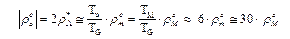In the field of the Macronucleus, the disintegration of nucleons occurs also because an ultra-high nuclear temperature close to the critical value: TN @ 1013 K, generated periodically by a big black hole -according to CGT.

For a position with R > the Ru/2 of the cosmic body, the winds coming from "stellar cemetery" (S.C.) zone generates a pressure in the sense of slowing the Universe expansion, so we may approximate the Universe’s expansion law by the eqs:(59a) ve= tR = vM ×sin(pR/Ru); vM @ ke×c ; R £ RL= (1/6)Ru Û sin(πR/Ru) @ (πR/Ru);  (59b)in which the maximum value, vM @ ke×c < c, was considered as the maximum expansion speed, (a value: ke » 0.5 corresponding to the redshift of the quasar 3C295: vq = 0.46c).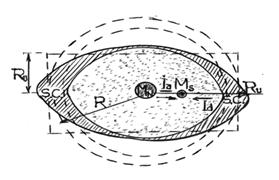Fig. 7. The Expanding Universe model.

According to the model, the Hubble law is valid in the zone of the local galaxy super cluster (Virgo) and its surroundings, and it may be regained from eq. (59a) by the condition (59b), i.e.: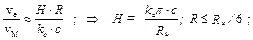(60a)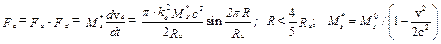(60b)

With the mean value: Ha =75Km/s×Mps, deduced by A. Sandage in 1958  and by a plausible value: ke » 0.5, it results from eq. (60a) that: Ru = 6.28x103 Mps, (27.3x109 l.y.) - of two times bigger than those deducted by the Big-Bang cosmological model-corresponding to an Universe filled with stars.

For a cosmic body Ms , the expansion force has the form (60b) in which Fa represent the accelerating force - given by the pressure of the stellary winds (mainly-etheronic winds) coming with the radial mean intensity Ia from the zone of the expansion center and Fd represent the decelerating force, given by the pressure of stellary winds coming with the radial mean intensity Id from the C.S.-zone and by the resistance force to advancing, given by the density of the sub-quantum and quantum medium of the cosmic "vacuum", (giving an apparent mass variation).

The mass: Ms* represents the virtual mass given by the relativistic relation (18), the eq. (60b) being in accordance with the linearized form of the Einstein-de Sitter equation:

Rik - ½gik×R + L×gik = Tik = 0                 (61)

We may consider also that the intensities Ia and Id of the stellary winds generating the expansion force are given mostly by etheronic winds, acting upon the mass Ms, so the expansion force Fe results conform to eq. (16) of the gravitation force, the maximum value of Fe-force being given for R = Ru/4, by the eqn.: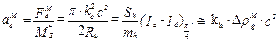(62)

With the gauge value: kh @ 27.4 [m2/kg] resulted from the theory, considering that: ke » 0.5, (vM » 0.5c), it results from eq. (62) a value:

DrgM @ 5.47x10-29kg/m3, and because that the mean etheronic density, rs*, which ensures the gravitational stability of the material structures in the intergalactic space must be with at least two size order bigger, it results that :

rs* > 102×DrgM = 5.47x10-27kg/m3> rm @ Wm×rc @4x10-27 kg/m3,                 (63)

so- corresponding to the mean "dark energy" density value deduced in accor­dance with cosmological observations :

rs* = rL @ 1.2x10-26 kg/m3.

For a pair of quantons, in our galaxy (R=Rl), because the very small quanton radius, the gravitonic component: rgh(mh ) which gives the G-value by eqs. (25)-(26) may be considered approximate equal to the value Drg(Rl;G), i.e:

Drg(Rl,G) = DrgM·sin(2pRl/RU) » rgh(mh) = 6.2x10-30kg/m3, so GM corresponds to: GM(RU/4) = 8.8G(Rl) and to: Rl = 1.8x10-2RU - for the position of our galaxy, Milky Way.

The increasing of the expansion force Fe until the maximum value FeM is explained in the model by the increasing of the R-depending number of dark energy sources which generates the Ia(R) intensity , (i.e. with pulsatory antigravitic charge), contained by the SU(4pR2) sphere of Universe, (eq. (57)).

The recently observed distribution of quasars in the Universe sustains the previous explanation looking the "dark energy" provenience. The estimated value for rL* gives an important effect of "radiation aging" which may explain the Olbers paradoxe and which contributes to the total redshift effect, according to the equations:

D En= h×ni - h×nf = Ff×DR = ½kh×mf×rs×c2×DR = ½kh×rs×h×ni×DR                  (64a)

nf = ni×(1 - ½kh×rs×DR); za = Dn/nf = ½kh×rs×DR/(1-½kh×rs×DR);                 (64b)

Considering the position of the local supercluster of galaxies (Virgo) at RV = Rl » 1.8x10-2RU, it results from eq. (64b) the condition to receive photonic radiation from the margin of the stellary Universe, considered at:

RM = ¾Ru, according to the model:

Dn/ni<1 Þ rsc<2/kh×DR =3.77x10-28 Kg/m3; (DR =RM–Rl »RM ; kh=27.4) ;         (65)

From eq. (65) it results the conclusion that- because that we have: rs* > rM @ 6x10-28 kg/m3, we cannot receive photonic radiation from the margin of the stellary Universe, with: rs* @ rL @1.2x10-26 kg/m3 resulting that the maximal distance DRc from which we can receive photonic radiation is given by:

Dn/ni = 1 ÞDRc = 2/ kh×rs*= 6.08x1024m = 6.4x108 l.y.= 2.36x10-2 Ru.         (66)

Comparing the za redshift with the redshift given by relativistic Doppler effect:

zr= [Ö(1+v/c)/Ö(1-v/c)] -1» H×DR/c,                               (67)

with: rs @ rL @ 1.2x10-26 kg/m3, for: DR =10-2Ru, we have: za »0.424/(1-0.424)= 0.73 and zr» 0.016, so it results that the redshift za given by the "tired light" effect is much greater than the redshift zr given by the Universe inflation- in accordance with the conclusions of "sub-quantum kinetics" theory of Paul LaViolette which showed (1987) that the tired-light model fits observational data better than the "expanding Universe" model, as showed also Tolman, (1985). So, the red-shift of 1.4 or higher, observed to many visible galaxies may be explained by eqs. (64) as "aging radiation" effect, explaining similarly also the high value of the red- shift observed to distant supernovae (of Ia type).

It results also that the high value of some quasars redshift:  z = 4, (vm= 0.92c -1986) and z = 6.3, (vm = 0.92c -2001) is given by an intense "tired light" effect generated partially by the density of quantum and sub-quantum medium, increased by the strong magnetic field of a rotational (Kerr - type) "black hole" and by its gravitational attraction .

Also, the proposed inflation scenario of CGT based on the pulsatory antigravitic charge model, eliminates the hypothesis of "inflaton", (quantum-particle of the inflation field).

Because that the density of the un-compensed etheronic winds, Drg, acts as a gravitic flux: DjDrgc2, generated by a total mean gravitic charge density: rGt = (rm+ ra)R of the Universe mass, Mu(R), by eqs. (56) and (60), neglecting the value of space radiation density rR , it results also that: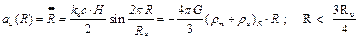(68)

The variation of the mean total gravitic charge density of the Universe mass, Mu(R), given by the Universe expansion, results from eq. (68), in the form: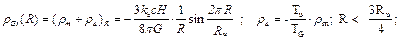(69)

Also, because that for v(R) » H×R, (R£Ru/6), we have: ½ra| = 2rL*» 6rm, it results that: Rl £ Ru/6 Þ Tu » 6·TG(Ru/2).

For: H =Ha = 75Km/s×Mps, (), and the eq. (68) becomes:; (ra » -6rm)      (70)

so: rL*» 3rm » 1.27x10-26 kg - in accordance with the known determined value: rL » WL×rc = 1.2x10-26 kg.

The equality: rm(Ru/2) = -ra(Ru/2) resulted from eq. (70) is explained with the eq. (54):(71)

The value ra º 0 corresponds in the model to the "thermal death" of the Universe’s stars.

4.2. Exotic stars

4.2.1. The Gravistar as Genesic Structure

Relative to the Protouniverse structure, it results in CGT, by the similitude principle, an anisotropic model of "gravistar"- considered as a hard-core rotation ellipsoid of "primordial dark energy" containing vortexially generated "dark photons" and "dark particles"- formed as Bose-Einstein condensates at distinct levels of density. This possibility is argued also by the known model of "gravastar" with very cold core formed by a "dark energy" fluid, which may create Bose-Einstein condensate in the outer core, , but which suppose an already existent central "black hole".

In the proposed model of hard-core gravistar, , the "gravitational vacuum" region specific to a "gravastar", not exists, the quasi-stability of the hard-core deformed ball of "dark" energy, forming a relativist vortex of quantons: Gm = 2pr×vc, (vc®c), being given- in the model, similarly to the electron case, by a quantum potential, VG(r) which- in the volume with exponential variation of the proto-dark energy, satisfy the stability condition in agreement with a NLS equation of (28)- form, in which: i ħ×(y/t) = 0 , (null variation with time of rc(r) by expansion or contraction).

If pc (r) = (rcvc)r is the impulse density of the relativist quantonic component of the "dark energy", forming the gravistar vortex: GG = Gm+ Gs of quantons and sinergons, a dmp- mass of vortexially formed "dark" photons or of "dark" particles, is attracted until a tangential vpt -speed for which the dmp- particle remains at the same r- distance from the gravistar’s center.

This GG-vortex is resulted initially as a small perturbation which may generates electronic neutrinos by quantons confination and thereafter- massive neutrinos with own magnetic moment, given by the GG- vortex, at values of the dark energy density: rc ³ra0 = 5.17x1013kg/m3, (equal to those of a proto-electron, i.e. –of a cold barrel-like electron).

According to CGT, it results that the force which ensures the gravistar forming is generated as in the electron’s genesis case, i.e. - by the quantum pseudomagnetic potential of the pseudo-vortex: Gs = 2pr×w, (Ö2c ³ w > c), being given by the QG(r) potential, (eq. (30)):

QG = -mc×BS(r) = -mc×k1×rs*c = -h/2, which maintains also quantons with vct » c to a vortex-line lv=2pr, but supplemented by the gravitic force , Fgs.

The gravitation force Fgs necessary for maintain quantons with vc = c to a given vortex-line, lv, in particular -at the surface of the star’s hard-core- considered as compact cluster of neutrons (of mn-mass), for which:

rg(R*) = rg0(a)×(M/mn)×(a/R*)2= 1837rge×(R*/a) »1.61x10-11×R*[kg/m3],             (72)

is given- for a gravitation constant G*» G and rge(a) » 1.24x10-29 kg/m3, (CGT), according to eq.(13):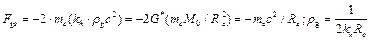(73)

With mc= ms and the values: rs »10-28 m and rh/rs »103, obtained in CGT, it results that rg0(R*) necessary by eq. (73) for maintain vortexed sinergons to lv- vortex line, is smaller than rg0’(R*) necessary for maintain quantons at the M0- core surface, for which the eq. (73), gives:

rg0’ = 1/(2khR*) » 1.8×10-2/R*, (rg0» (rh/rs) rg0 ; kh= 27.4)                (74)

So, the M0 hard-core is formed gradually, by quantons confining and thereafter by "dark" photons confining, the vortex Gc of quantons being formed after the pseudovortex Gs of sinergons, with the contribution of the QG –potential.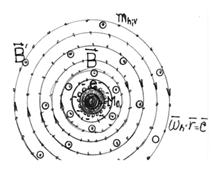Fig. 8. The Gravistar model.

It results that the M0 hard-core growing increased the density of vortexed sinergons and quantons at its surface until values of "frozen" photons and of "frozen" electrons genesis: rLv » 3.7x104Kg/m3; rLe » 5x1013Kg/m3, which corresponds by eq. (14) to specific values of ratio: (M0/R*2) = rg0×(khc2/G*).

Inside a zone DR = R0 ¸RG of quantum equilibrium, i.e. having the entropy per quanton:

eh(r) = g×(kB/ ħ)×Sh(r), (Sh(r)=2pr×mcc ; g ~1/M0 ,  )  (75)

the variation of the "proto-dark energy" impulse density results- in the model, by the similitude principle, as in the electron’s case, i.e.- with exponential variation of the quantons energy (forming dark photons in the gravitic and the pseudomagnetic field of the gravistar -eq. (26)), with: rc ~ e-(r-R*)/h in the zone with formed dark photons of the gravistar- considered as having the effective RG radius, and with: rc~ r-2 in the outer zone, (in the evanescent part).

The dark photons were formed vortexially, according to the model, by the xB vortex-tubes of the hard-core magnetic field having the induction: Bm(r) ~k1Ñrsc, with: rs(r) » rc(r), initially being formed the vectorial photons.

These xB vortex-tubes favored the negatrons forming, which are vortexially more stable than theirs antiparticles, explaining the spontaneous symmetry breaking in the particles genesis process and theirs magnetic moment anomaly: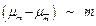.

It results also that the formed electrons gives a negative electric charge to the gravistar’s kernel.

The dynamic equilibrium between the pseudomagnetic and the centrifugal potential, is realised for vortexially formed vectorial photons, mv , with mc­­BS and a tangential speed vf according to the equations: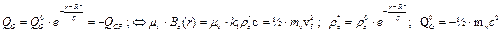(76a)

with: vf2= v02×e-(r-R*)/h. For: rc*=rc0(R*)→ ra0(a) = 5.17x1013 kg/m3, it results: BS→2x1012 T ; mv =3×1010mh= 4x10-36A/m2. Generally: (vf2)e = 2mvk1rc*c/mv , the value of r* resulting by eq.:

FGM = ÑQG(r*) = -h-1×QG(r*) = mvvf2/r* = -2QG(r*)/r*; Û r* = 2h                                            (76b)

r* being a pseudomagnetic equivalent of the gravitational Schwarzschild radius.

The values: h, rc* and r* increases gradually, proportional with R*, according to the sub-solitons forming condition, .

Adding the gravitic force, (eq.(73)), for a formed pseudoscalar photon: mf = (mv-`mv), the radius re of "photon sphere" of the gravistar results by the dynamic equilibrium equation:

mvvf2/re = (mv/mh)×(Fg(re) + FGM(re)) , (FGM(re) = (-h-1×QG(re, mh) = -(h/2h) e-(r-R*)/h)                     (76c)

(Fg(re) and FGM(re) acting over a single quanton).

The equations (76) explains the gravistar hard-core gradual growing:

-in the field of the gravistrar’s magnetic moment: Gm(r) = 2prvc , the pseudoscalar "cold" photons and the vectorial photons with lower speed, were attracted with oriented mc to the M0- hard-core surface, where generated- at a specific rL- density, cold electrons and thereafter- ultracold nucleons, formed as Bose-Einstein condensate of photons , respective- of quasi-electrons, the M0- hard-core becoming in time a magnetar-type star, a supernova or a rotational "black hole" with (super)dense neutronic shell, but with a density:

rn << rcM = 3x1023 kg/m3.

Thereafter, by the gravitostatic Fgs force, a formed "black hole" may generate in the neutronic shell, nucleons destruction with emission of g-rays and neutrins, at: rc= rs*>>rn0= 4.6x1017kg/m3, transforming the gravistar into a GRB star of magnetar type or into a quasar- by the antigravitic pseudocharge, conform to eqs. (12), (43) .

The maintaining of the formed photons inside the gravistar’s volume is conditioned also by a dynamic equilibrium equation on the tangetial direction, similar to eq. (22), with w = Ö2c, i.e:

rr(r)×vf2= rs*(r)×(w - vf)2; with: rs*(r) = rs0×e-(r-R*)/h; vf2= v02×e-(r-R*)/h                                               (77)

For r>>R*, eq. (77) imply: rr(r) » 2rs0, i.e- a condition which may not be satisfied, according to the sub-solitons forming condition  which imply the necessity to ensures a negentropy value specific to the quantum equilibrium (75).

It results that the conditions (74); (77) are realised only at M0 hard-core surface, when: rr(R*) = rs0(w/v0-1)2, resulting also the condition of M0 -hard-core growing:

rs0(R*) ³ rr(R*) > rs0(w/c-1)2» 0.17×rs0(R*)  (78)

The transformation of the gravistar into a "black hole" results conform to eq. (76c).

So, according to the model, if G*³G, the gravistar hard-core which is transformed into black hole is initially a neutronic rotational star growed initially from a superdense "seed-core" with density rn*®rh» 3x1023 kg/m3.

The conclusion of the electron/proton genesis as B-E condensate of 3K-photons is sustained also by the fact that the confining temperature for the electron forming, results by B-E equation of value: Tc @ 3.31×ħ2/3/(m×kB) » 6x10-10K, for n »re/mv, i.e. - bigger than the quantons temperature:          Th » 5x10-11K..

It results that the cold genesis of "dark" photons and of "cold" elementary particles was possible in the Protouniverse’s period by gravistars forming which- in this case, may explains also the supposed "big-bang" scenario of the Universe genesis by a fractalic process of multi-gravistars forming and by theirs transformation into supernovae and into (micro) quasars containing a "black hole" of "magnetar" type, in the first stage.

In a similary way it may be explained also the Multi-universe with a structure of expansionary pseudo-bubbles, for example, in accordance with the conclusions of the Fractal cosmology, (L. Pietronero, 1987, ).

So, according to CGT, the Protouniverse’s period had some Eras specific to:

1.  the gravistars forming from gravistaric "seeds", ( nm;nt- heavy neutrino with GG-vortex; electronic centrol clusters );

2.   the dark photons confining and the formation of "dark electrons";

3.   the "dark particles" forming and confining; -the "atonium" states forming;

4.   the forming of "black holes" and of micro/mini-quasars.

The hypothesis of an Universe’s Macronucleus forming, having a macro-vortex of "dark energy", may be also sustained by the conclusion that- locally, the biggest gravistar deter­mined the attraction of other locally formed gravistars in its magnetic field, forming a super-magnetar with super- black hole, by the transformation of the gravistars into magnetars.

4.2.2. The Magnetar as Source of Particles Genesis and/or Acceleration

The existence of magnetars as neutronic stars converting rotational energy into magnetic energy to more than 1011 tesla B-induction  and of microquasars: sources of high energy with only 103km diameter , sustains indirectly the previous conclusions regarding the particles cold genesis in the Protouniverse’s period by gravistars forming, which indicates that the electric charge of the magnetars is a negative charge, given by negatrons.

Also, extrapolating the eq. (1) of the theory for bigger m- mass of stable/quasistable particles, it results two supermassive quasistable particles, formed in a very strong magnetic field as clusters of ½Kv pairs of degenerate quasi-electrons:

mY = me×Kv» 5x(1014¸1016) eV and mZ = mP×Kv» 9.4x(1017¸1019) eV                                            (79)

This theoretical result, for mZ»9x1019eV, explains the zetta-particles, (1020-1021MeV), detected by AGASA ("Akeno Giant Air Shower Array", ).

The forming of supermassive particles, (mP >1010GeV/c2), in the primordial Universe is deduced also by unified gauge theories of elementary particles , but as formed "at hot".

According to CGT, after the gravistar’s transforming into a black hole, it may obtain also- in particular conditions, a pulsatory antigravitic charge, by matter Þ energy conversion (~10%M0- for supernovae), with pulsatory emission of light, of gamma quanta and of neutrins, which may generate- in particular cases, also laser emission, as a known Eta Carinae supernova.

It is know -in this sense, that the known magnetars, such as the SGR 1806-20 magnetar, are tipically soft gamma repeaters, which suggest- in CGT, also the existence of a periodically matter destruction, generating pulsatory antigravitic (pseudo)charge- according to the theory.

If the formed black hole is of rotational (Kerr) type, it is possible to exists also a very strong magnetic field, characteristic to a magnetar type star, which may be given- according to CGT, by an electric charge of the magnetar surface or by a sub-quantum and quantum macro-vortex:

GBH = GA + GB of sinergons, generating the magnetic A-potential (eq.(10)) and the magnetic induction, B , (eq.(9), with a density variation conformed to those of a gravistar, (eq. (77)).

Relating to this case, in CGT are explained microphysically  also some magneto-electric and magneto-mechanic effects, such as: Einstein – De Haas, Barnett, Joffe-Kapitza, Aharonov-Böhm, Hooper-Monstein effects and the London’s equations of superconductivity, by the hypothesis of the gravito-magnetic nature of the magnetic potential, A , in particular- by the conclusion that the modification of the canonic impulse of a moving electron: dpe = ±e×A is given as a real impulse modifying by the dynamic sinergonic pressure of the GA –vortex :

Ps = ½ rsc2 , .

For example, by CGT, we may conclude that the Aharonov- Böhm effect, consisting in the electron phase changement at passing through a magnetic field of null B-induction but non-null magnetic A-potential, with an additive phases: dle =h/dpe with dpl = ± eA, is done by a speed/impulse modification by a (quasi)electric field: Eq = dA/dt considered as being generated by the electron entering in the field:        dA = ±A in a time dt in which this field Eq ~ rsc2acting over the electron, determines an impulse variation:

dpe = me(vf – vi) = mea×dt = e× Eq×dt = e×dA; Eq = k1rsc2 » dA/dt ; dA » A                              (80)

with:

A = ½ B×r = ½ k1rB(r)×c×r = ½ k1rc(r)×vc(r)×r = ½ k1rs(r)×rmc                                          (81)

Eq acting as a gravito-electric field over a gravito - electric e-charge and giving a final impulse:

pf = mevi + dpe = mevi ± e×A; dpe = e×dA » ± e×A                                                        (82)

An identic effect is obtained also in the magnetic field of a star, but also for the electrogravitic charge, qG, of a neutral particle- according to CGT and to the Schiff-Barnhill effect, by the force: Fq= qG ×Eq , phenomenon that may explain the possibility of the particles accelerating in the field of a pulsar or of a „black hole" type star- considered in the actual explicative model as a particularly gravitational effect generated  near the event horizon, .

4.2.3. Stellary Pulsating Sources

According to CGT, a strong enough „black hole" having an source of matter in its proximity, such as a matter accretion disk or a ordinary star, will generate- at the black hole’s surface, a high pressure/density and matter destruction, at T³ 1011K, (close to TN »1012¸ 1013K), releasing the particles’ energy: mc2, in the form of quantonic winds and gamma and neutrinic fluxes (given as pairs of centrols of the released degenerate gammons which -in normal state, constitutes the Np neutral cluster of nucleons).

Also, according to CGT, the sinergonic (etheronic) winds generated to the gammon®neutrino conversion, (eq. (43) –when also the sinergonic vortexes of the paired electrons are reciprocally annihilated), contributes to the mean value rL of the „dark energy" as fluxes of quanta of an antigravitic (pseudo)charge of the „black hole".

Writing the electric field energy of an electron in the form: ÎE =½ a×Fe(a) = mec2, for: Fea = -e2/4pe0a2 and FeN = -G×me2/a2, it results for the electron’s (electro)gravitic energy, that:

ÎG = ½ a×FeN(a) = me2G/2a; Þ ÎE/ÎG = ra0/rg0=2ac2/meG = 4x1042,                                    (83)

i.e.- the gravitic field energy of the me-gravitic charge is of ~1042 times smaller than the etheronic energy contained by the sinergonic GA - vortex of the particle’s magnetic moment:      Îs = mec2/2.

If the mean flux of relativist particles which are destroyed is a little higher than the critical value F0a which cancel the MG - gravitic charge of the "black hole", the generated antigravitic charge of BH: Ma = -kaMG with ka>1, will determine the rejection of the matter and will cancel its cause, decreasing the Ma value at ka< 1, and because that the induced acceleration and deceleration of particles, corresponding to ka<1 or ka >1, is realized quickly but gradually, it results that the antigravitic charge Ma of the BH is a pulsatory, oscillating charge, which not impede its growing and not affect its stability. So, the hypothesis of a BH antigravitic charge is non-contradictory.

Also, if the central black hole is of rotational (Kerr) star of magnetar type, with a strong magneto-gravitic field: VMG ~ r-3 (eq. 10), this field can exceed the resulted antigravitic field: Vat ~ r -1, under a critical limit, rl , continuing in this way the cause of the Ma antigravitic (pseudo)charge generation:

r < rl Þ VMG (r) = V0MG(r0/r)3 >Vat0(r0/r)2           (84)

It results in this case, by CGT, that the quasars, the galactic centers, some supernovae or/and hypernovae or also some magnetars with BH, may have a pulsatory antigravitic charge- conclusion which is in concordance with some astropysical observations, such as:

a)  In the case of Quasars:

It is considered that the power of quasar results from the accretion disk of a central supermassive black holes, that are believed to exist at the core of all galaxies and which can convert on the order of 10% of the mass of an object into energy- compared to 0.7% for the p-p chain nuclear fusion. But because that even the light cannot escape from the black hole’s field, it results the conclusion that the escaping energy is actually generated outside the event horizon, by gravitational stresses and immense friction on the incoming material, .

But this hypothesis is not enough fitted with the fact that- for create a luminosity of 1040 watts (the typical brightness of a quasar), a super-massive black hole must consume the material equivalent of 10 stars per year.

So, the hypothesis of CGT regarding the pulsatory antigravitic (pseudo)charge, may explain better the quasar’s energy.

It is deductible also that the period t of the radiation emission is inversely proportional wispecific to a neutronic star,th the mass of the central BH, (a bigger mass generating faster the critical pressure, pc ).

The previous explanation is in concordance also with the next astrophysical observations:

- the OVV blazars (compact quasars with optical violent variability) such as the quasar 3C279 has luminosity variations with 50% even in a day ;

-was observed also a periodically varying luminous quasar : PSO J334.2028 with a period of light variation of 542 days, caused by a specific mass accretion rate, .

-was observed quasars in pairs but also in triplets and even a quartet of quasars ,  ;

-the X-rays emission from Cygnus X1, considered as being a "black hole", has repetitive variations, (quasi-periodic oscillations) and g-rays emission in the very high energy band, E>100GeV, observed in the same time with the hard-X-rays, suggesting a causal link;

-there were some suggestions that quasars were made of some hitherto unknown form of stable antimatter which may explain their brightness.

b)  In the case of a Hyper- or Super- nova:

Generally, it is considered that the releasing of gravitational energy by matter falling towards a massive black hole is the only process known that can produce high power continuously, in the case of quasars, but stellary explosions–supernovas and gamma-ray bursts - can do likewise, for a few weeks.

- In the case of a collapsar, i.e.-a hypernova which produces a BH after the explosion, it is considered that if the star is rotating quickly enough, then the fallback to the black hole will produce relativistic jets whose energy, transferred into the ejected shell and will render the visible outburst substantially more luminous than those of a standard supernova, the jets containing high energy particles and gamma rays and generating x-ray or gamma-ray bursts of several seconds or longer, corresponding to long-duration gamma-ray bursts; but the model do not explain the short-duration gamma-ray bursts.

-Also, in 1966 Colgate and White  calculated that neutrinos carry away most of the gravitational energy released by the collapse of massive stars, (the case of Type Ib and Ic and Type II supernovae) because the proton- electron combining, with the forming of a neutron and an electronic neutrino at the stars collapse, at densities specific to a neutronic star, (1017 kg/m3), but resulting that a second and more important neutrino source is the thermal energy, of ~1011K, of the newly formed neutron core, which is dissipated via the formation of neutrino–antineutrino pairs of all flavors, , the theory being confirmed in 1987, when were detected the flux of neutrino emitted by SN 1987A.

We observe that the Colgate-White theory is in concordance also with the relation (43) of CGT by which we may suppose that- at the considered very high temperatures of the neutronic matter of the collapsed star: ~1011K , (which is close to those of particles deconfining experimentally determined:

Td» 3x1012K, ), a quantity of neutrons is transformed into g-rays which- by eq. (43), are partially transformed into neutrins, these radiations being characteristic especially to collapsars, to Ic-hypernovae and to GRB –sources, (particularly, to pulsars and to magnetars).

- Is is known also- in accordance with the explicative model of CGT, that exists also GRB sources emitting short gamma-rays bursts, of about 2 sec. period, with un-clear origin and cause, because that the gamma bursts are succeded of X-rays flashes after few minutes or few hours, suggesting the particles emission from a primary object like a neutron star swallowed by a „black hole".

Another hypothesis considers as cause of the phenomenon a neutron star with „black hole".

This hypothesis is closest to the CGT’s conclusion looking the generation of a pulsating antigravitic (pseudo) charge of a strong black hole according to eq. (43), by nuclear reactions at T > 1011K.

The long and ultra-long gamma-ray bursts are considered also as effect of nuclear matter collapse, particularly- generated by a „black hole" over a „white dwarf".

-There are also models which considers a newly formed magnetar instead of a BH, as cause of the long GRBs, .

This hypothesis may be, partially, in concordance with the CGT’s model because that –according to CGT, it may exist also magnetars formed by a "black hole", particularly- of Kerr type B-H.

Also, from eq. (47) of CGT looking the particles lifetime, it results that a „black hole" must be enough „cold" for attain a density:

rBH > rn0= 4.6x1017kg/m3, (of the impenetrable nucleonic volume), because that a double density: 2rn0, for example, may be assimilated with the density of a hexa-quark particle: 3x{qa(2/3) + qb(-1/3)}, which is un-stable even  at T®10-10K, in accordance with eq. (47).

So, a „black hole" with rBH>rn0 must be of „magnetar" type, according to CGT.

5. Conclusions

The possibility to explain the fundamental fields: gravitic, electric, magnetic and nuclear, by equations of the ideal fluids, as being generated by the impulse density of field quanta, may be considered– in our oppinion, a strong argument for the CF-prequantum model of particles of the theory, describing the fermion as ideal un-disturbed chiral soliton cluster in the ground state: T®0K, with determined parameters, in a Galileian relativity -like in the scale relativity theory of Nottale , which predicts- like in our theory, the natural apparition of some structures by spontaneous self-organising and the existence of a relation between the electron’s mass and charge.

At T>0K, in perturbative conditions, the prequantum particle becomes quantum, as in the case of chiral soliton electron which at T>0K becomes pseudosperical by spin precession, without the change of its spin value .

The conclusion of CGT regarding the electron’s cold genesis as "freeze" photons confining in a super-strong magnetic field, is indirectly sustained also by the recent experimentally creation of a "super-photon", i.e. a Bose-Einstein condensate of photons, which emitted yellow light in the moment of its creation, .

This experimental result sustains indirectly also the revised model of photon of CGT, with inertial mass equal with its spinorial mass, in our oppinion.

Also, the possibility to "freeze" light inside a crystal, experimentally proved , may be explained by the revised vortexial model of photon, by the hypothesis of ring multiphotons forming in the magnetic field of the electrons or/and of the atoms, (i.e. a n×hn ring photon around an e-charge).

The theory shows also that the classic model of nucleon with incorporate electron(s) may explain also the spin and magnetic moment values by the conclusion of a density-dependent degeneration of the electron’s magnetic moment and a null spin of the electronic neutrino, the total spin conservation law being respected only in the moment of the degenerate electron releasing.

This phenomenon invalidates the Yukawa’s hypothesis of the nuclear field mesonic theory, which results as being a formal theory, without prequantum correspondent at T®0K.

The conclusion is not contradictory because that a soliton-like particle is an open system in the quantum and subquantum vacuum, according also to the particle’s thermodynamics of de Broglie and explains the fact that- at the proton transformation by K-electron capture, the electron’s spin and its magnetic moment are not transmitted with the normal Se , mB, values to the formed  neutron.

So, according to the theory, all elementary particles may be formed „at cold", by a ‘gravistar’ or by a magnetar type star, in a „cascade vortex" process, as clusters of quasielectrons, from the „primordial dark energy" composed of etherons and quantons.

The use of a galieian-like relativity in CGT is justified also by the very low photon speeds obtained in the "stopped light" experiment, .

Also, the phenomenological model of expanding Universe, results in CGT with semi-sinusoidal variation of the expansion speed, with the "dark energy" which generates the expansion given by an antigravitic (pseudo)charge of stellary energetic sources with central "black hole", given by matter®energy conversion, with the releasing also of the sinergonic energy.

The considered structure of the particles magnetic moment, with a quantonic vortex inducing the B-induction vector by a sinergonic vortex which is the energetical cause of the magnetic potential A, may explain also microphysically the main magneto-electric and magneto-mechanic phenomena and may be verified also by new possible experiments.

A general field equation compatible with the theory is of Maxwell-Proca type: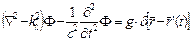with kl » mq×c/ħ; g = -Q/e, (mq- the quantum mass; Q-the electric or electro-gravitic charge) –for the electro-magnetic and the gravitic field and: kl2»(2mni/ħ2)×VP0; g = 0, Vp ~ F, with:VP = kn×|F|2 = VP0(o)×e-r/h, for the nuclear field.

The exposed phenomenological approach of CGT does not propose an enough unitary equation of these three basic fields , but explains naturally the particles genesis and their fundamental interactions, by the same basic concept: the "proto- dark energy", as a vortex cascade mechanism.

A more complete and accuracy approach and the set of possible validation experiments, may be the subject of a further work.

References

1. M.Arghirescu - ‘The Cold Genesis of Matter and Fields’, Science Publishing Group, (2015).
2. T.H.R. Skyrme, Proc. Roy. Soc. Series A260 (1961) 127 G.S. Adkins, ‘Chiral Soliton ‘, ed. by G.S. Adkins, Add. - Wesley, (1988); O.L. Battistel, Doctoral thesis, Instituto de Fısica da Universidad de Sao Paulo, Brazil, (1994).
3. E. Mottola, P.O. Mazur‚‚Gravitational Condensate stars: An Alternative to Black Holes’ arXiv: gr-qc/ 0109035 (2001).
4. I. Şomăcescu - ‘L’éléctromagnetisme et la gravitoinertie en une theorie unitaire’ International Conf. of Gravitation, G.R.11, Abstract Book, Stockholm, 27 feb. (1986); E.M. Kelly -‘Vacuum electromagnetic derived from the properties of an ideal fluid’, Nuovo Cimento, 11, 32B, March (1976).
5. Georges L. Lesage-‘Lucrece newtonien’, Memoires de l’Academie royale des sciences et belles lettres de Berlin, Berlin (1784) 1-28.
6. R. P. Lano- ‚Gravitational Meissner Effect’, arXiv: hep-th/9603077 (1996); N. I. Pallas, L. Sofonea- Book of International Congres of Mathematicians, Helsinki (1978).
7. M. Agop, C. Gh. Buzea, B. Ciobanu - ‚On Gravitational Shielding in Electromagnetic Fields’, arXiv: physics/9911011 (1999);
8. Hector A. Munera - ‘A semiclassical Model of the Photon based on Objective Reality and Containing Longitudinal Field Components’, Myron W. Evans- „Modern Nonlinear Optics", Ed. John Wiley&Sons Inc., (2002) 335-385.
9. G. Hunter, R. L. P. Wadlinger - ‘Photons and Neutrinos as Electromagnetic Solitons’, Physics Essays, Vol. 2 (1989) 158-172.
10. R. M. Kiehn - ‘The Falaco Effect, A Topological Soliton’, Talk at 1987 Dynamics Days, Austin, Texas, jan. (1987); R.M. Kiehn-’Coherent Structures in Fluids are Deformable Topological Torsion Defects’, IUTAM-SIMFLO Conference at DTU, Denmark, May 25-29, (1997)
11. G. Hunter, L.P. Wadlinger et. al, ‚Gravitation and Cosmology: From the Hubble Radius to the Planck Scale’, „Fundamental Theories of Physics", Vol. 126, (2002), pp 157-166.
12. Weinberger, P., Philosophical Magazine Letters 88 (12), (2008) 897–907.
13. A. Cotton, H. Mouton, Ct. r. hebd. Seanc. Acad. Sci. Paris 141, (1905) 317- 349.
14. R. D. Chipman, L. D. Jennings, Phys. Rev. 132 (1995) 728; Greenberg, O. W., Yodh, G. B., Phys. Rev. Lett., 32 (1974) 1473.
15. K. N. Muhin – ‚Experimental Nuclear Physics’, Vol. 1-2, Ed."Atomizdat", Moscow (1974).
16. I. Sick - ‘On the rms-radius of Proton’, Phys. Lett., B 576 (2003) 62-63.
17. G. Adkins, C. Nappi, E. Witten - ‚Static properties of nucleons in the Skyrme model’, Nucl. Phys., B228 (1983) 552-566.
18. P. Constantinescu, „Hierarchized Systems", Ed. Acad. R. S. R., Bucharest (1986) 106.
19. V. P. Oleinik - ‘Nonlinear QDE for Self-acting Electron’, Nonlinear Math. - Phys. Rev., v.4, No1-2 (1997) 180.
20. W. Peschka- ‚Kinetobaric Effect as possible Basis for a New Propulsion Principle’, Raumfahrforschung, no 18 (1974).
21. D. L. Burke et al., ‚Positron production in multiphoton light - by light scattering’, Phys. Rev. Letters 79 (1997) 1626-1629; Physics Today 51, Feb. (1998) 17-18.
22. M. Brunetti et al., ‚Gamma-ray bursts of atmospheric origin in the MeV energy range’ Geophys. Research Letters 27, 1 (2000) 1595.
23. R. Jastrow-Phys. Review, 81 (1951) 165.
24. Y. Yan, R. Tegen- ‚N-N Scattering and Nucleon Quark core’, Science Asia, 27 (2001) 251.
25. Patent: US2482773.
26. Dan Rădulescu - Bulletin de la Société des Sciences, Cluj (1922).
27. The OPERA Collaboration (2011), ‚Measurement of the neutrino velocity with the OPERA detector in the CNGS beam’, ar Xiv: 1109.4897v1.
28. L. Wolfenstein- Phys. Lett., B 107 (1981) 77.
29. Shull Michael, Stern Alan, Astronomical Journ., Vol. 109, No2 (1995) 690-697.
30. Science et Avenir, No. 555, may (1993).
31. B. Rossi, D. B. Hall - Phys. Rev., 59 (1941) 223.
32. T. Schmidt, Z. Phys., 106 (1937) 358.
33. T. Lonnroth, Il Nuovo Cimento 110A (1997) 961.
34. W. Bauer, Proc. of 1st Catania Relativistic Ion Studies, Asicastello, Italy (1996) 23.
35. A. Săndulescu et. al - Nucl. Physics., 48 (1963) 345.
36. Shaw P. E., Davy N., Phys. Rev., Vol. 21, Ser. 2, Iss. 6 (1923) 680-681.
37. J. Kristian, A. Sandage, J. Westphal - Astrophys.Journal, 221 (1978) 383.
38. W. L. Freedman et al., Astrophys. J. 704 (2009) 1036; arXiv: 0907.4524.
39. C. F. C. Brandt, R. Chan, M. F. A. da Silva, P. Rocha, ‚Gravastar with an Interior Dark Energy Fluid and an Exterior de Sitter-Schwarzschild Spacetime’, arXiv: gr-qc/1012.1233v1 (2010).
40. Pietronero, L. ‚The Fractal Structure of the Universe: Correlations of Galaxies and Clusters’, Physica A (144): 257, (1987).
41. C. Kouveliotou, R. C. Duncan, C. Thompson, ‘Magnetars’, Scientific American Rev., Febr. (2003) 237.
42. I. F. Mirabel- ‚Microquasars as sources of high energy phenomena’ arXiv: astro-ph/0211085 v1, 5 Nov. (2002).
43. ‘Scientific American’, January, (1999)
44. Polnarev, A. G., Khlopov, M. Y. – ,Primordial Black Holes and the Era of Superheavy Particle Dominance in the Early Universe, Soviet Astronomy Journ., V.25 (1981) 406.
45. O.B. Zaslavskii, „Acceleration of particles as universal property of rotating black holes", arXiv: 1007.3678v2 [gr-qc] 15 oct. 2010; O.B. Zaslavskii, „Acceleration of particles as universal property of rotating black holes", arXiv: 1007.3678v2 [gr-qc] 15 oct. 2010
46. Thomsen, D. E.- ‚End of the World: You Won't Feel a Thing’, Science News (Society for Science & the Public), Jun 20, (1987), 131 (25):391,
47. Tingting Liu et al., ‚A Periodically varying Luminous Quasar at z = 2 from the Pan-Starrs1, Medium Deep Survey: A Candidate Supermassive Black Hole Binary in the Gravitational wave-Driven regime’, arXiv:1503.02083v1 [astro-ph. HE]
48. Irene, K.-‚Surprise Quasar Quartet Defies Explanation’, news.discovery.com., 14 may, (2015)
49. S. A. Colgate & R. H. White- ‚The Hydrodynamic Behavior of Supernova Explosions’, The Astrophysical Journal, 143 (1966) 626 ; doi:10.1086/148549
50. A.K. Mann (1997) Shadow of a star: The neutrino story of Supernova 1987A. W. H. Freeman. p. 122.ISBN 0-7167-3097-9
51. Metzger, B. et al.- ‚Proto-Neutron Star Winds, Magnetar Birth, and Gamma-Ray Bursts’. AIP Conference Proceedings „SUPERNOVA 1987A: 20 YEARS AFTER", 937, (2007), pp. 521–525.
52. L. Nottale‚ Scale Relativity and Fractal Space-Time: Applications to Quantum Physics, Cosmology and Chaotic Systems’, Chaos, Solitons& Fractals, Vol.7, No.6 (1996) 887-938.
53. Klaers, J., Schmitt, J., Vewinger, F., Weitz, M., Nature, 468, 546-548 (2010); Zeeya Merali, ‚Chilled light enters a new phase’, Nature, 24 Nov. (2010).
54. Heinze, G., Hubrich, C., Halfmann T., ‚Stopped Light and Image Storage by Electromagnetically Induced Transparency up to the Regime of One Minute’, Phys. Rev. Lett. 111, 038103 (2013);
55. L. V. Hau, S. E. Harris, Z. Dutton, C. H. Behroozi, "Ultra-slow, stopped, and compressed light in Bose-Einstein condensates gas", Nature 397, 594, (1999); S. E. Harris and L. V. Hau, Phys. Rev. Lett. 82, 4611 (1999).

 Contents 1. 2. 3. 3.1. 3.2. 3.3. 3.4. 3.5. 3.6. 4. 4.1. 4.2. 5.
Article ToolsAbstractPDF(766K)
Follow on us
PUBLICATION SERVICE
JOIN US
RESOURCES
SPECIAL SERVICES
ADDRESS
Science Publishing Group
548 FASHION AVENUE
NEW YORK, NY 10018
U.S.A.
Tel: (001)347-688-8931